# RS Aggarwal Solutions for Class 9 Chapter 9: Congruence of Triangles and Inequalities in a Triangle Exercise 9A

## RS Aggarwal Solutions for Class 9 Maths Exercise 9A PDF

The experts faculties at BYJU’S have designed the solutions with the aim of helping students perform well in the exams. The solutions mainly contain stepwise detailed explanations on how to solve the problems faster. The objective of providing solutions is to help students understand the concepts as it will be continued in Class 10 as well. The PDF format of the solutions train the students on ways to answer the question based on CBSE guidelines. RS Aggarwal Solutions for Class 9 Maths Chapter 9 Congruence of Triangles and Inequalities in a Triangle Exercise 9A are provided here.

## RS Aggarwal Solutions for Class 9 Chapter 9: Congruence of Triangles and Inequalities in a Triangle Exercise 9A Download PDF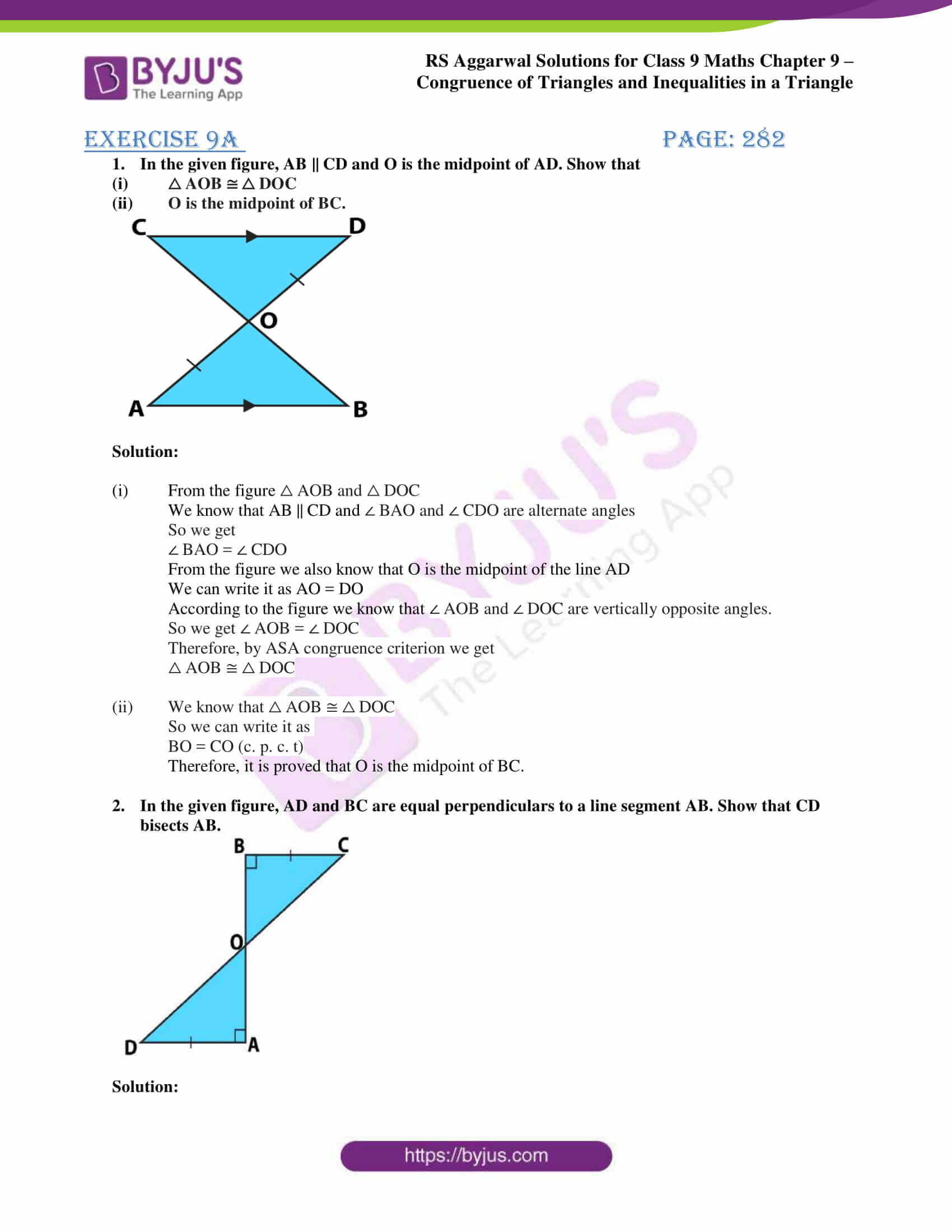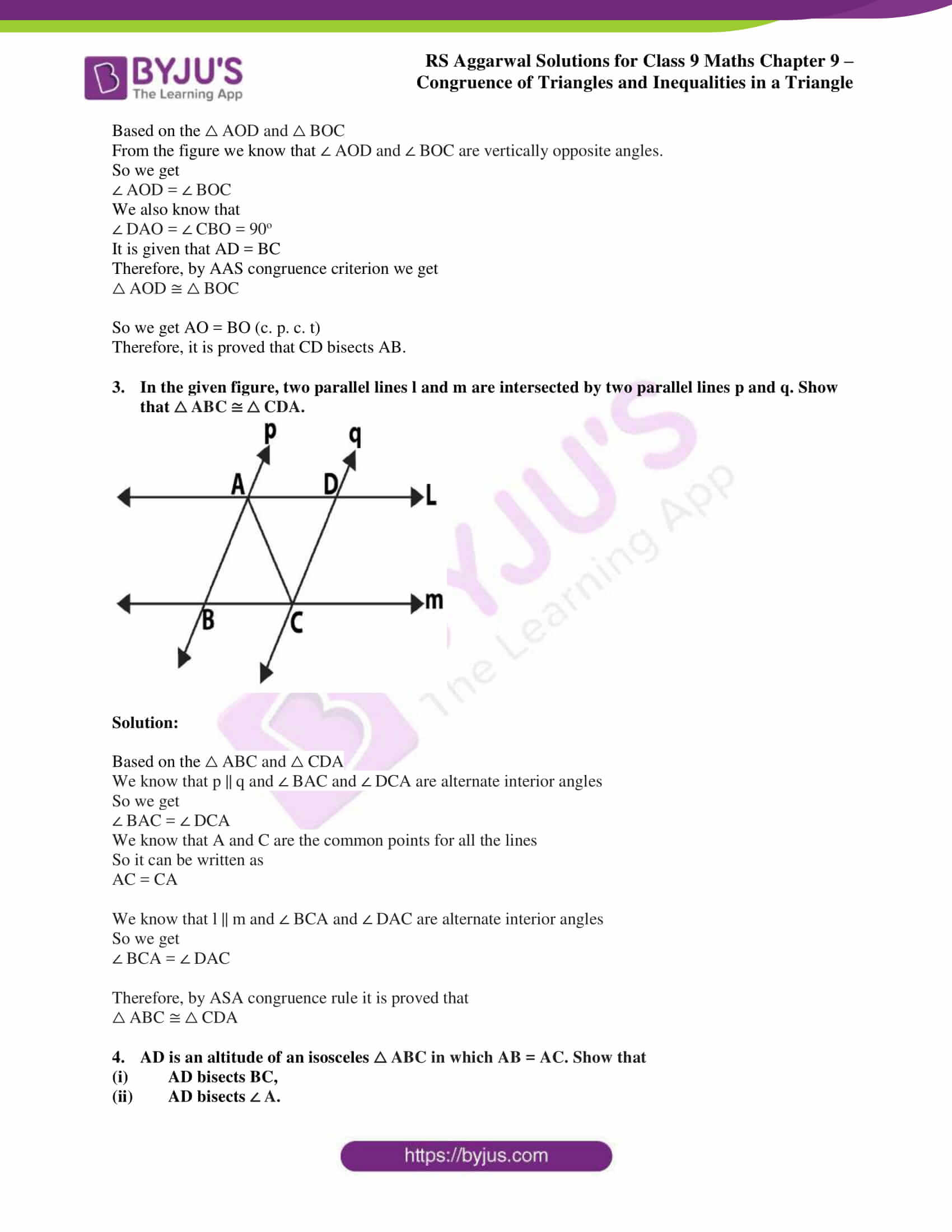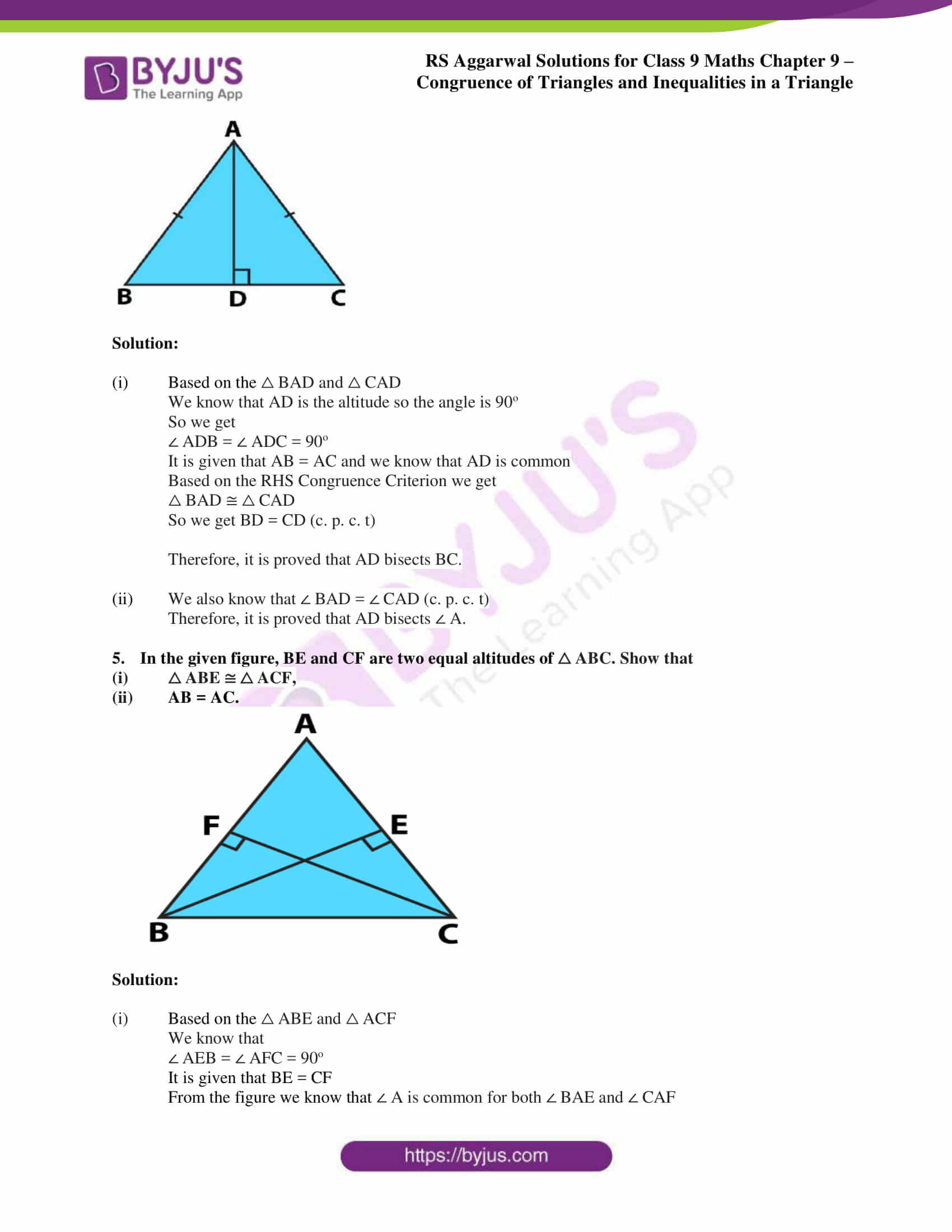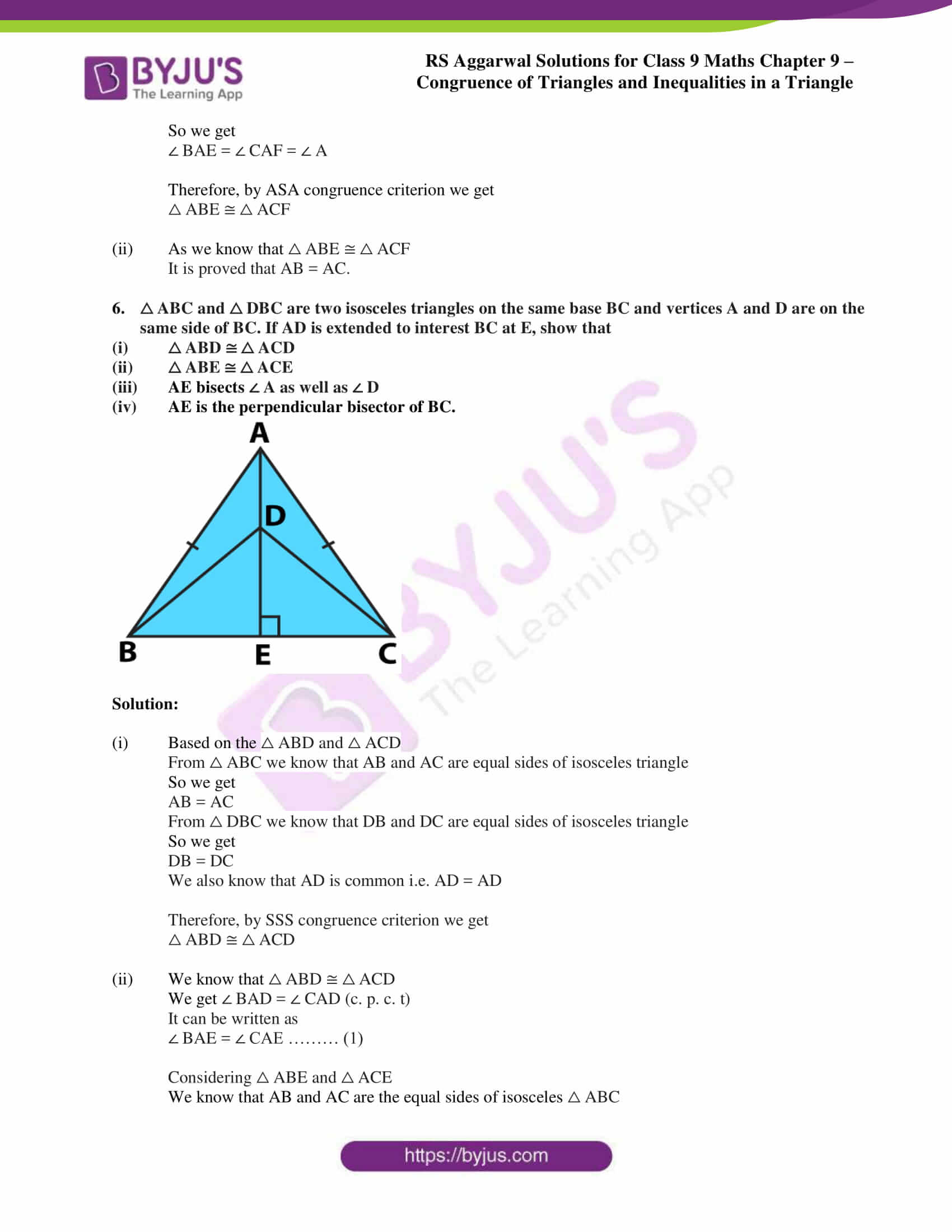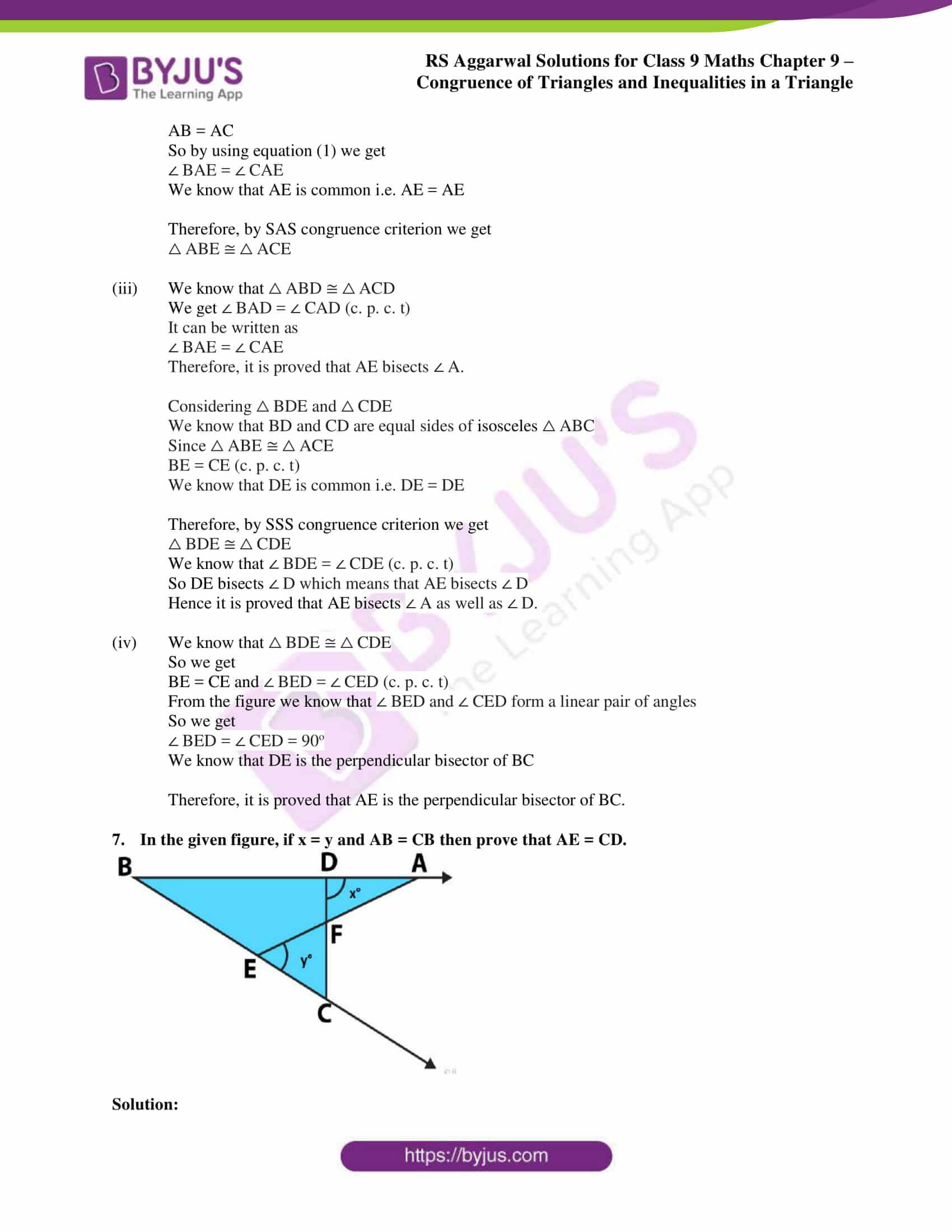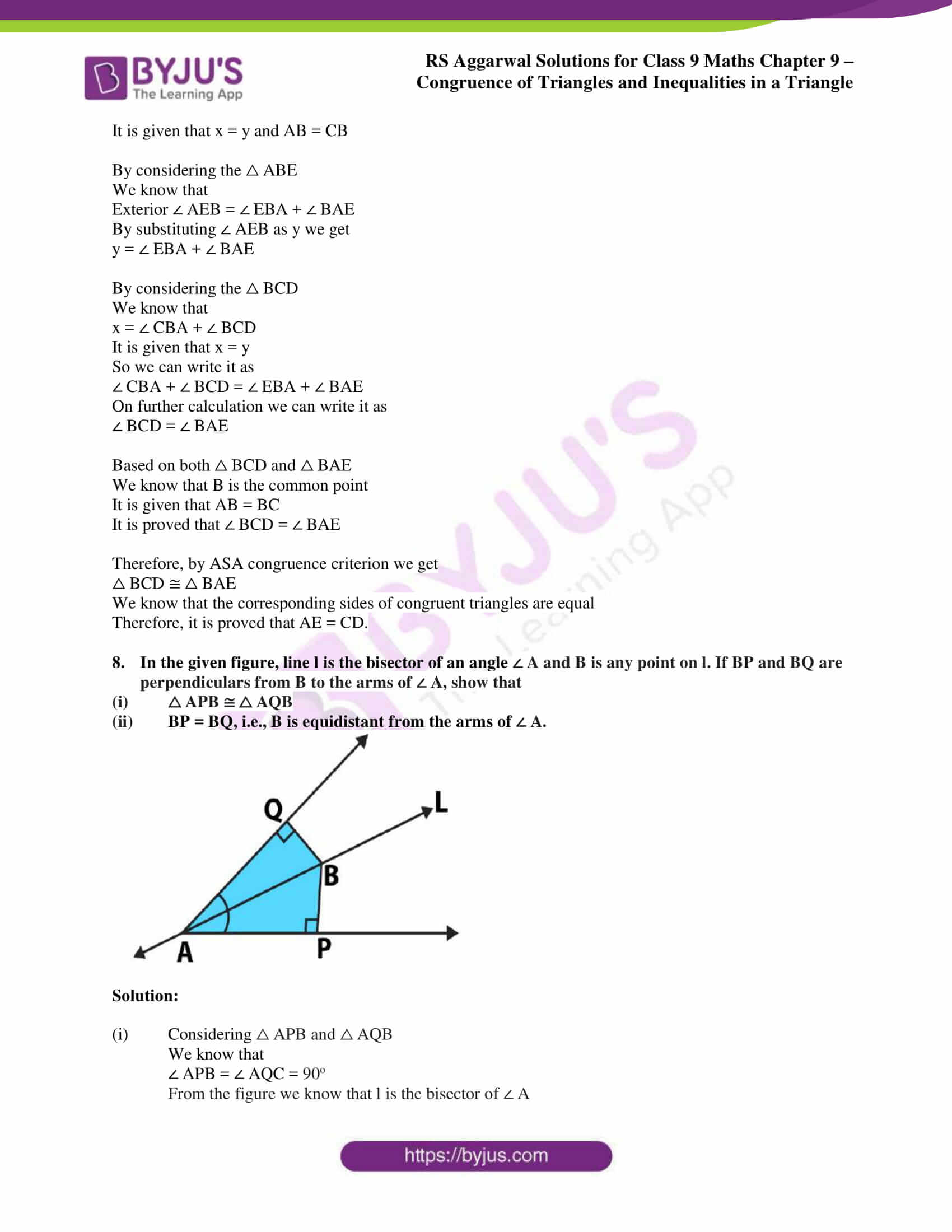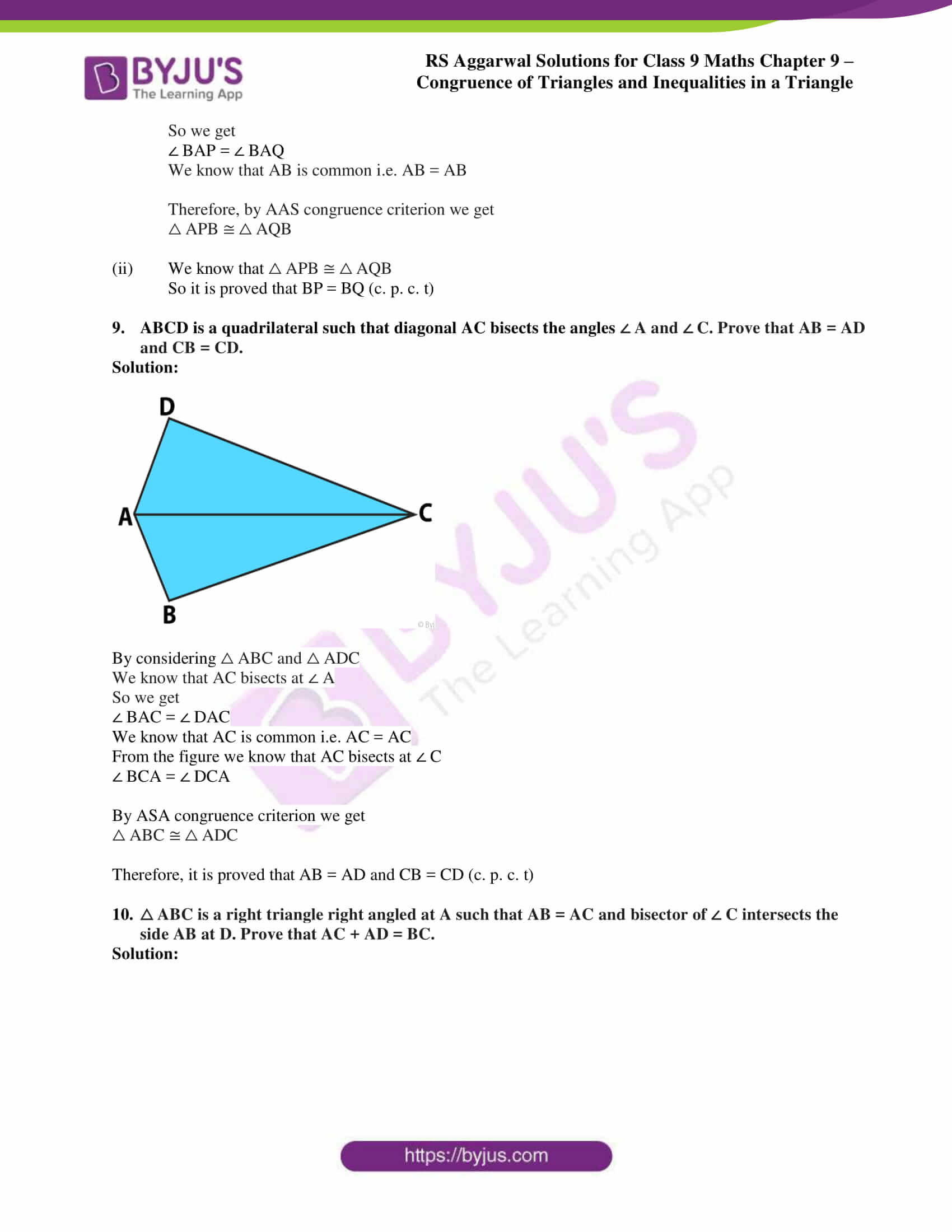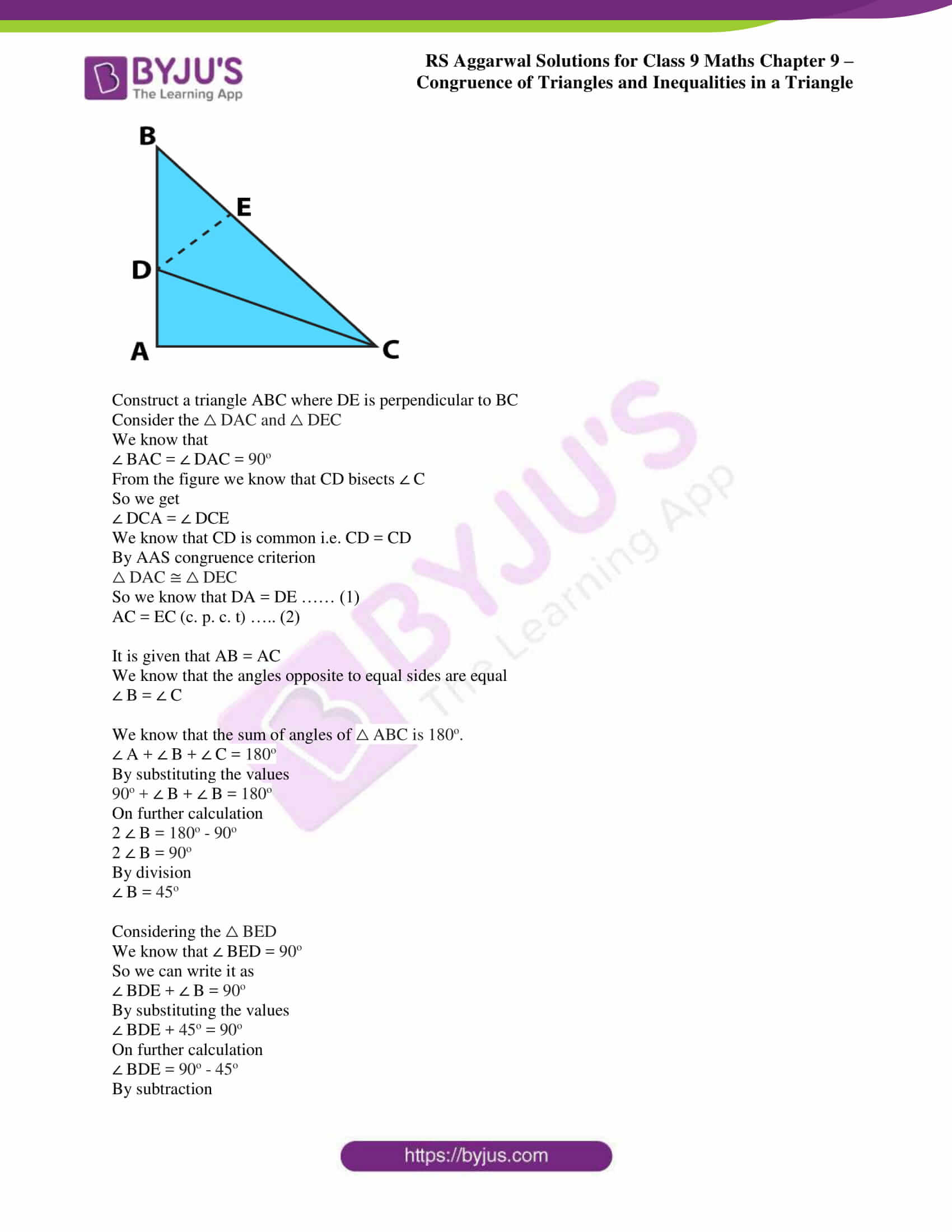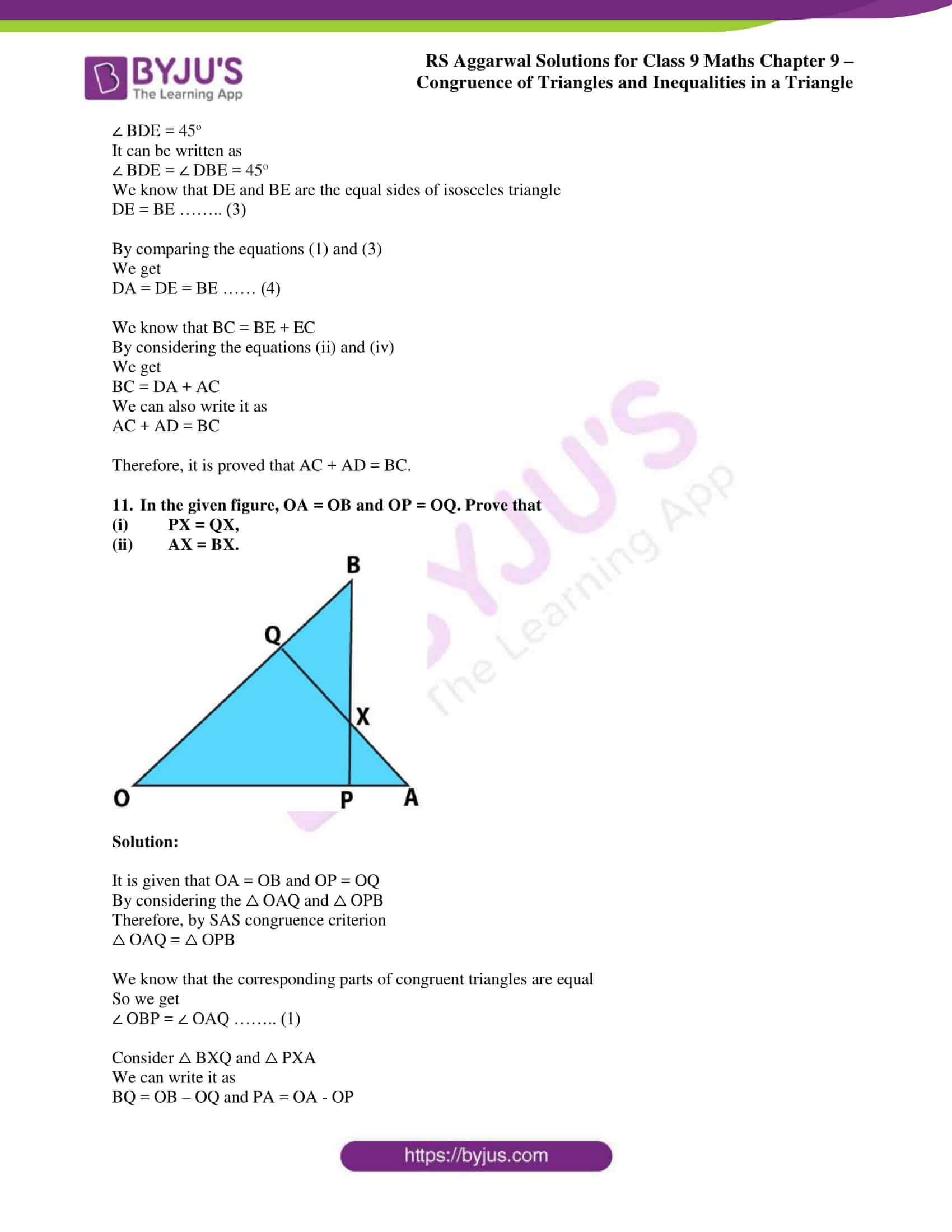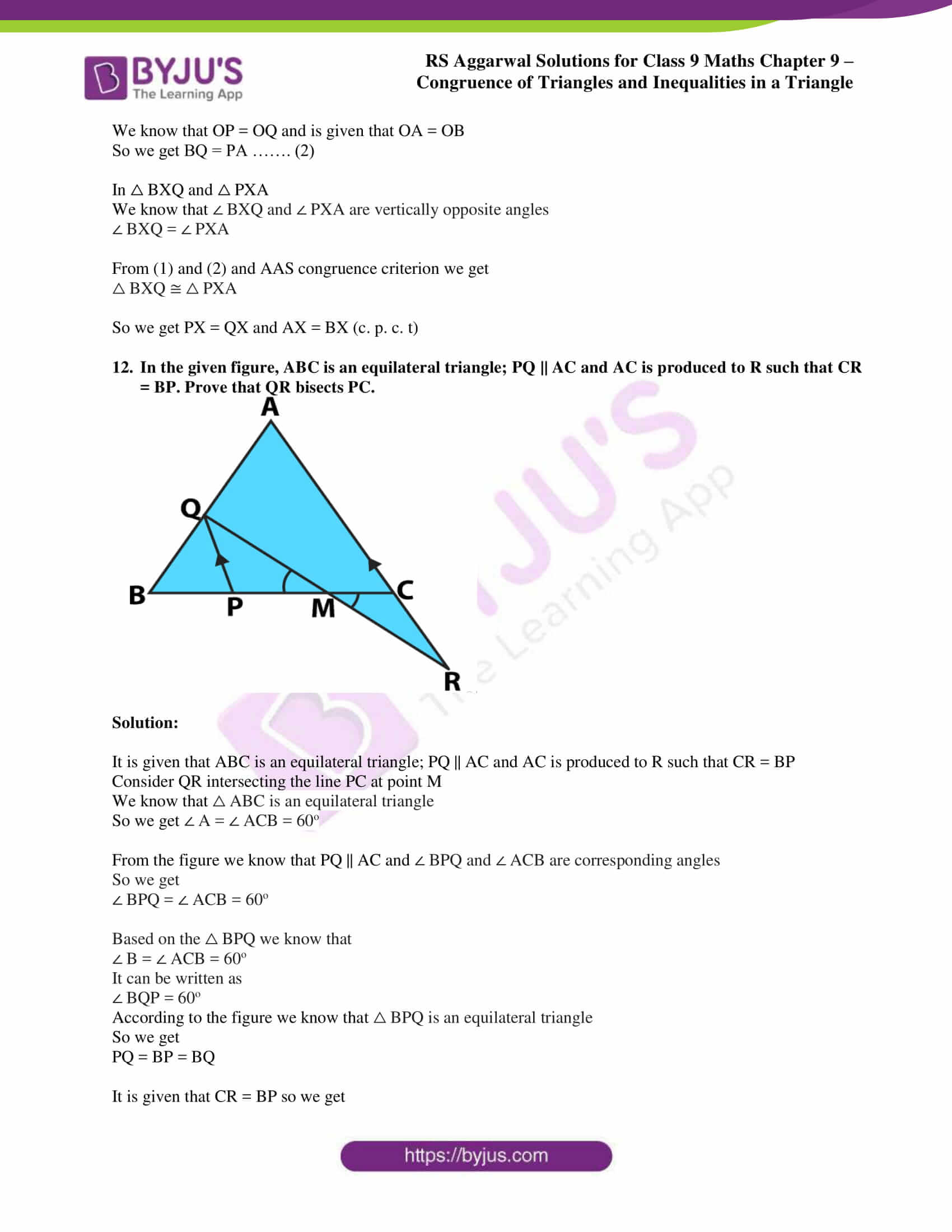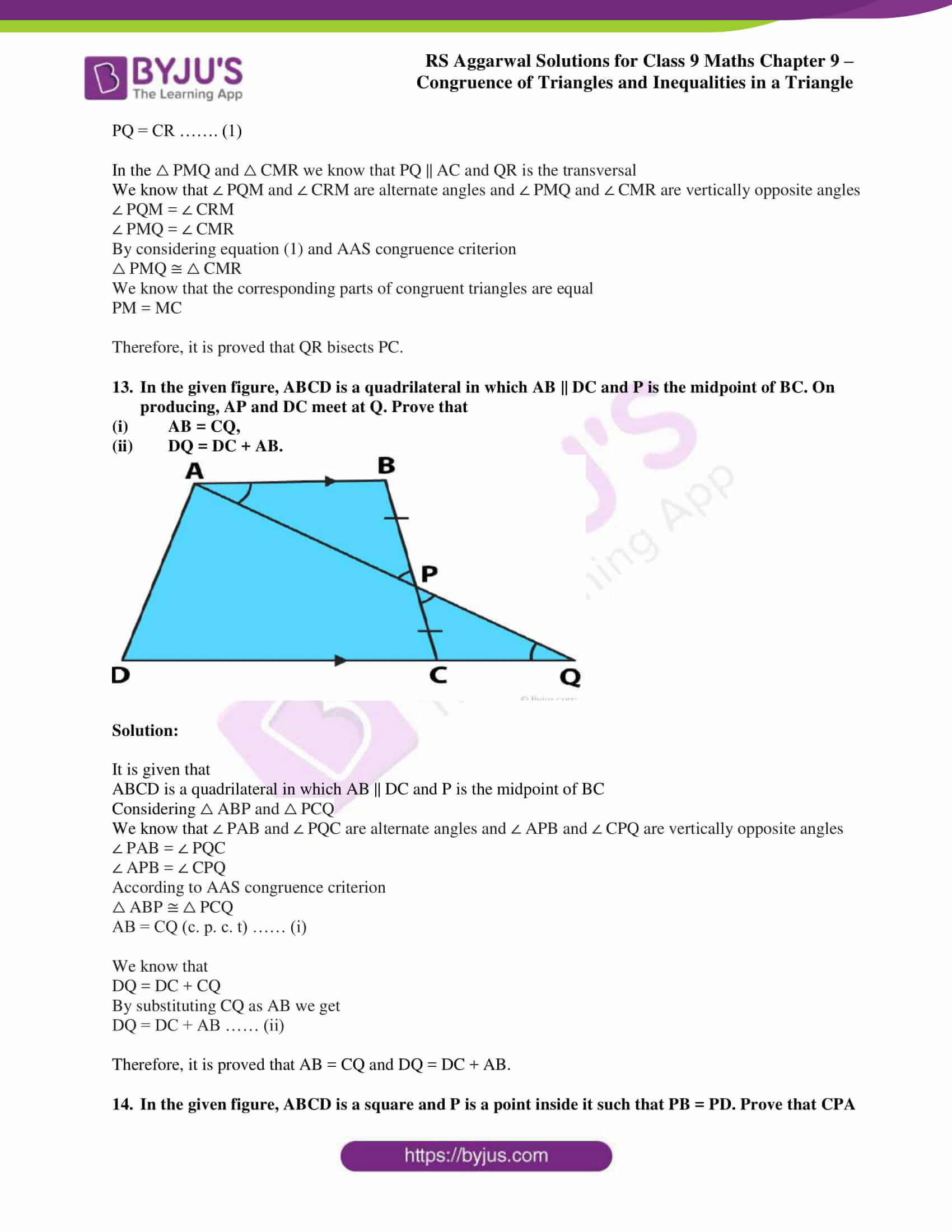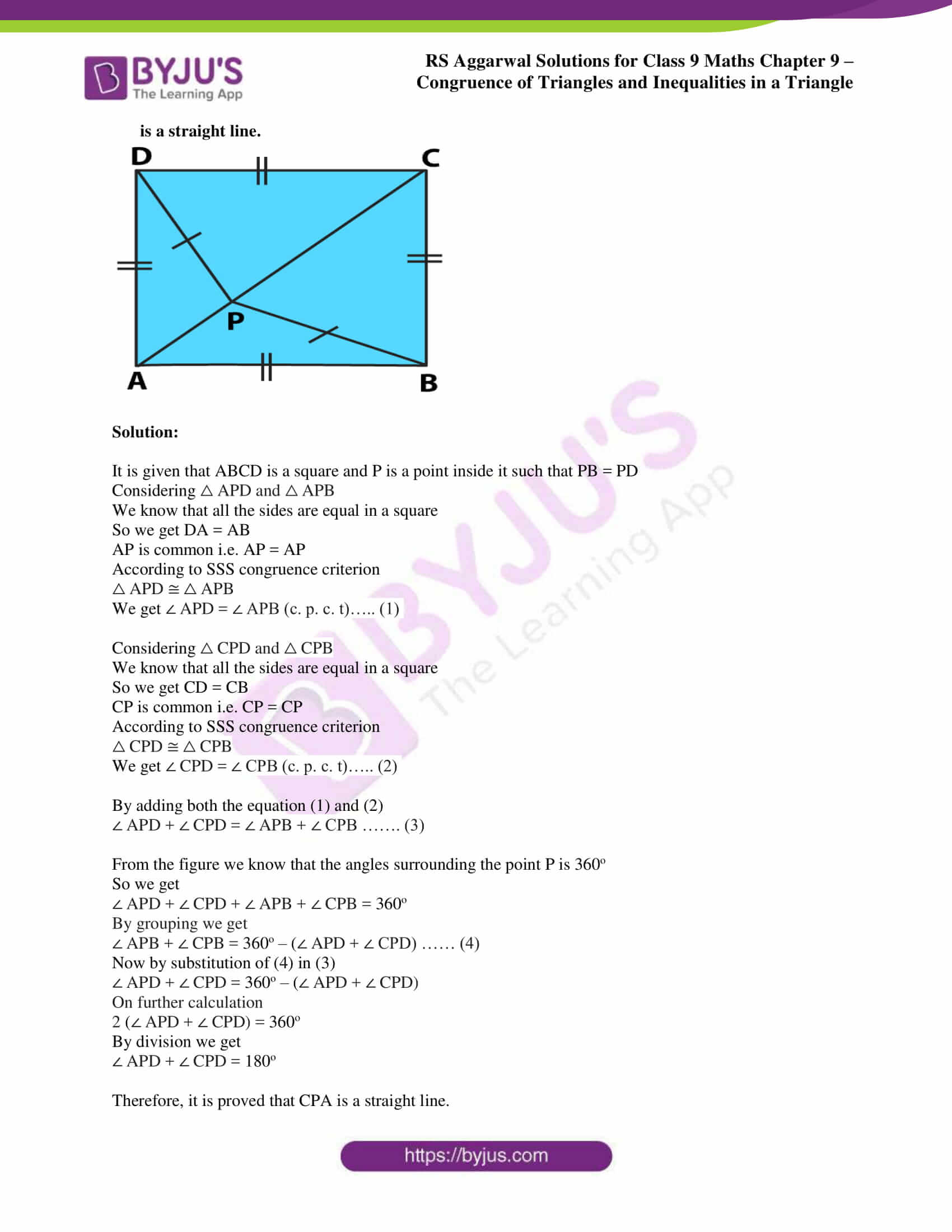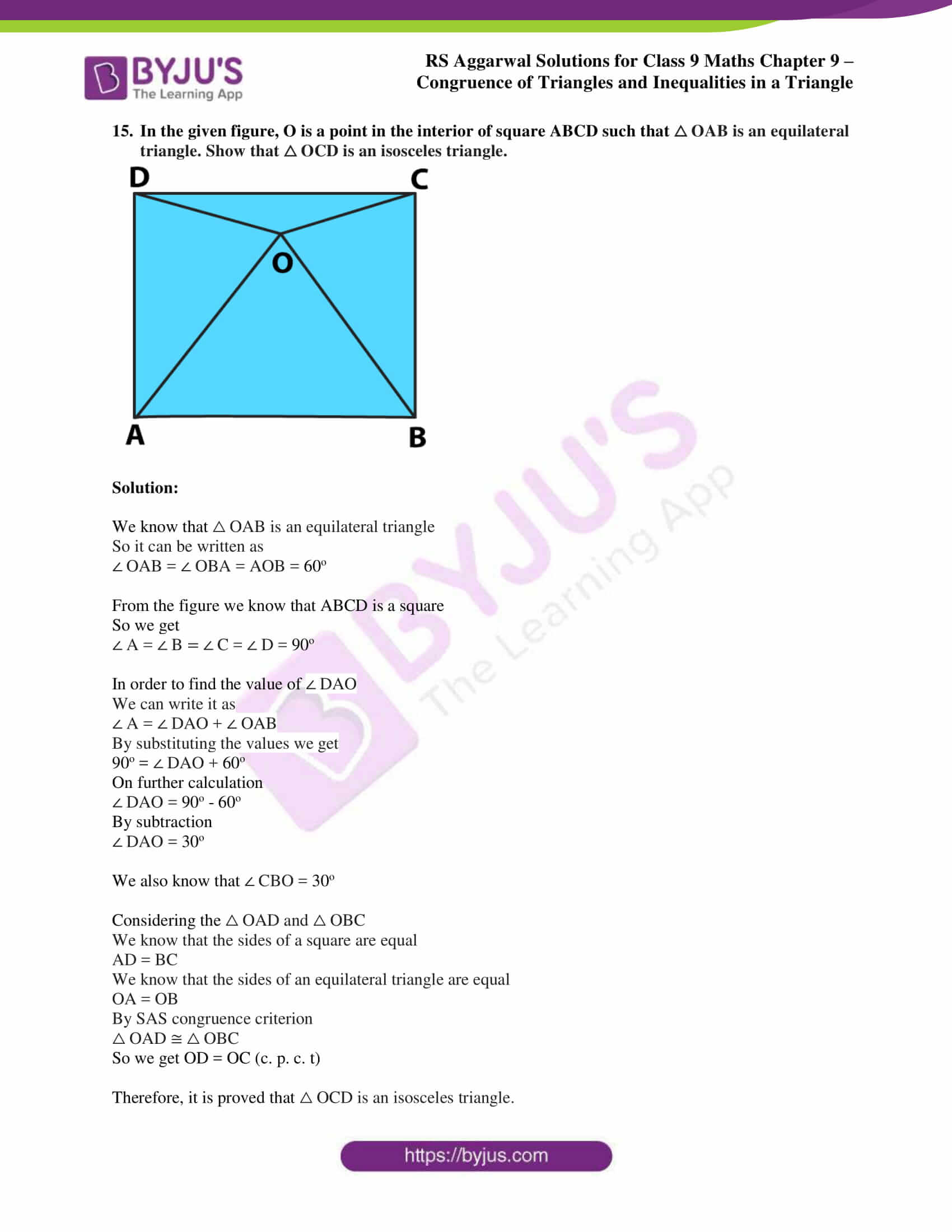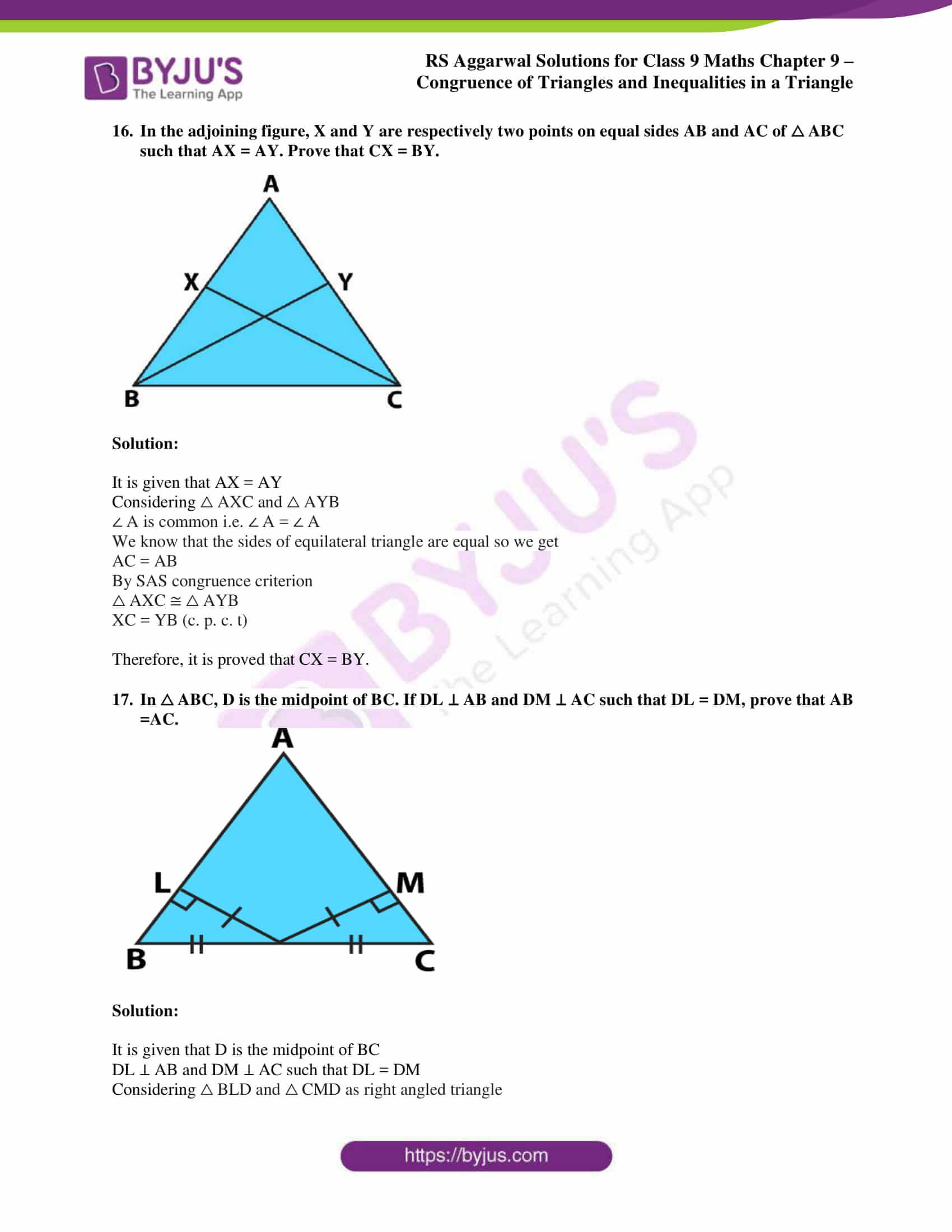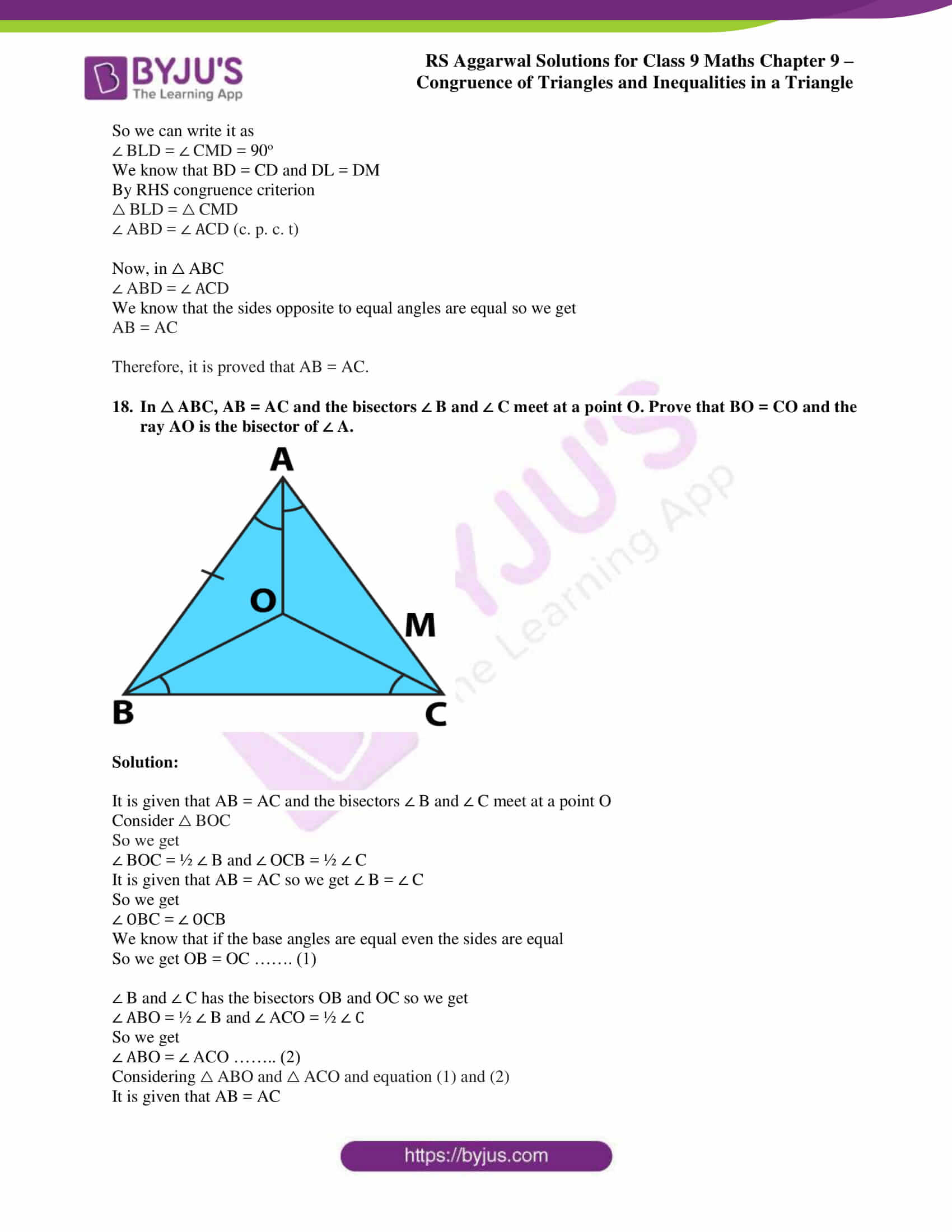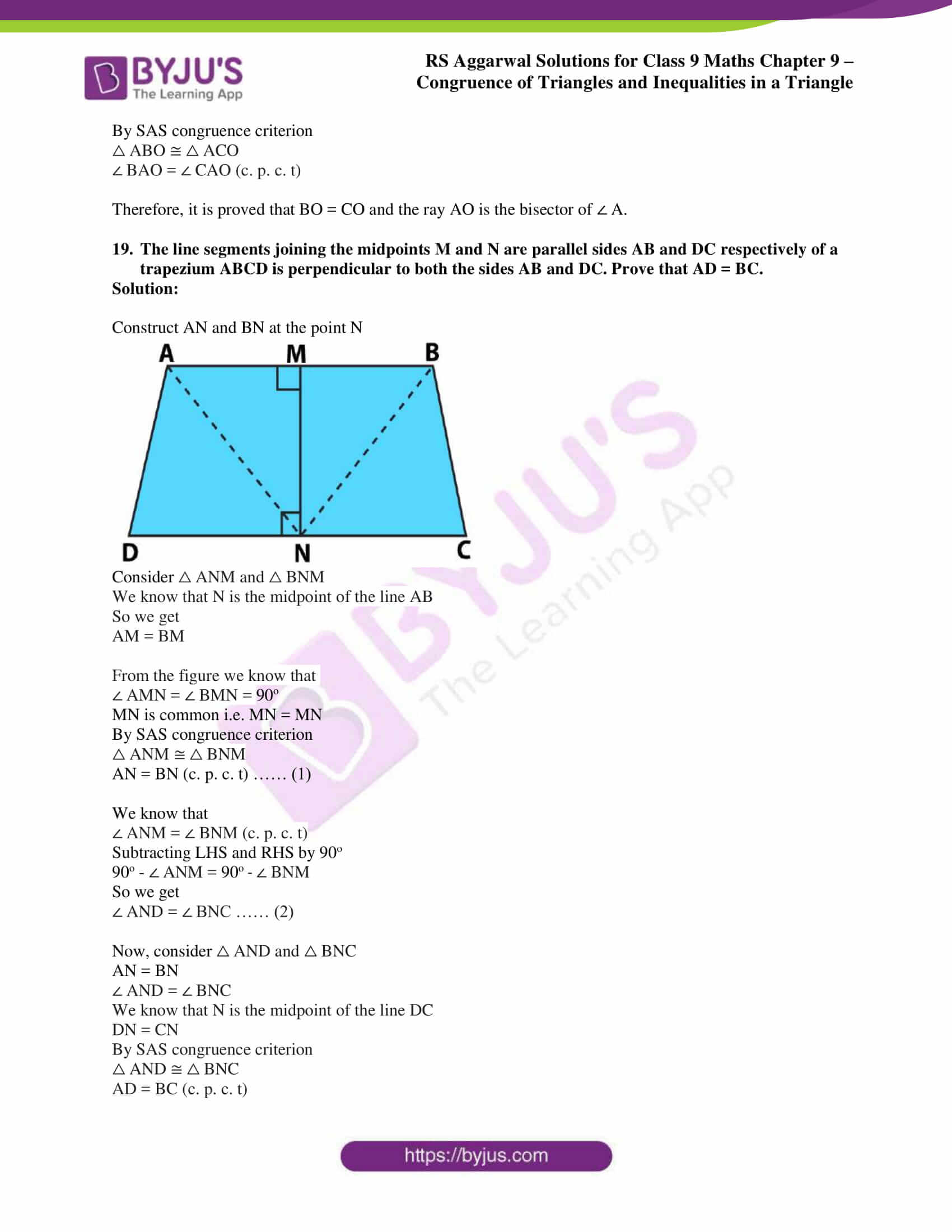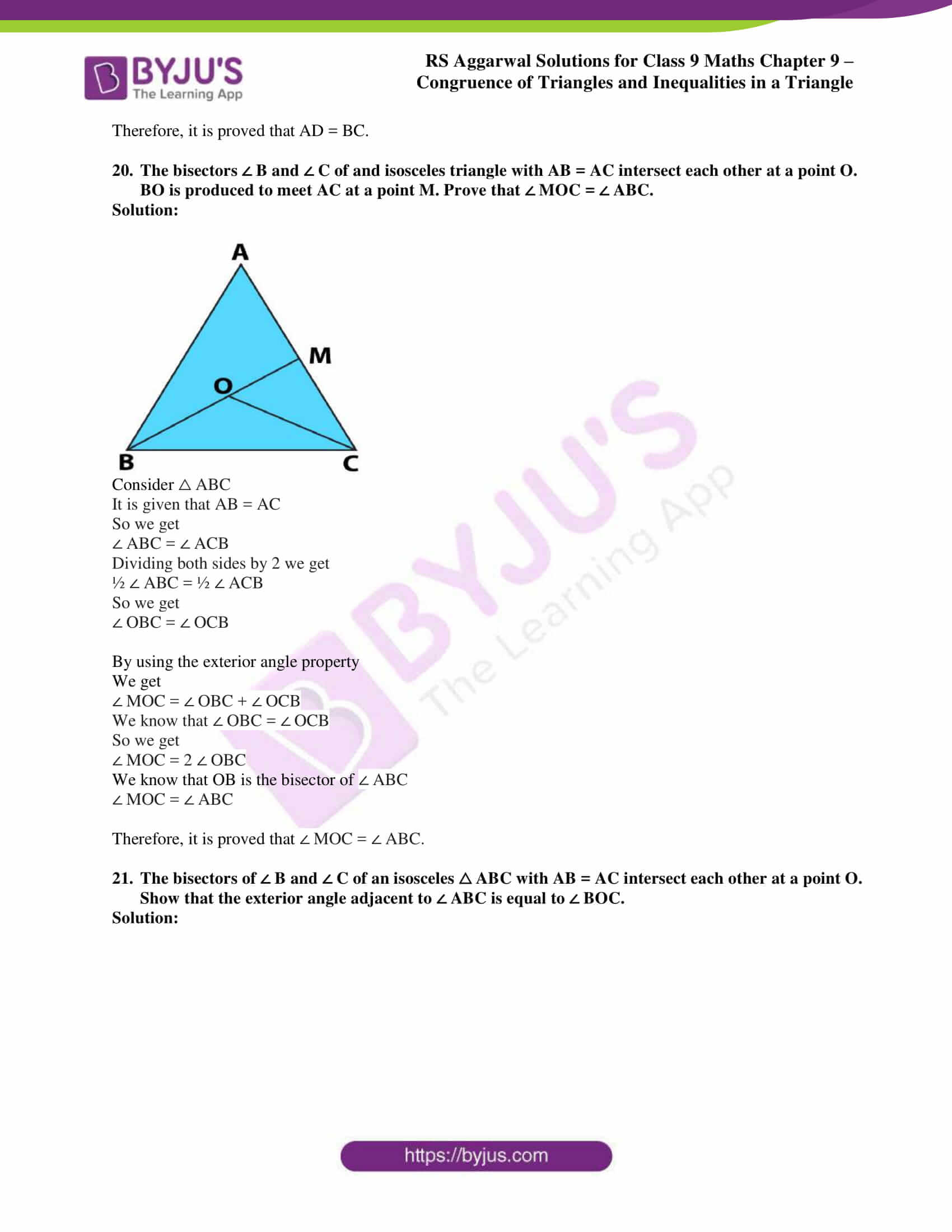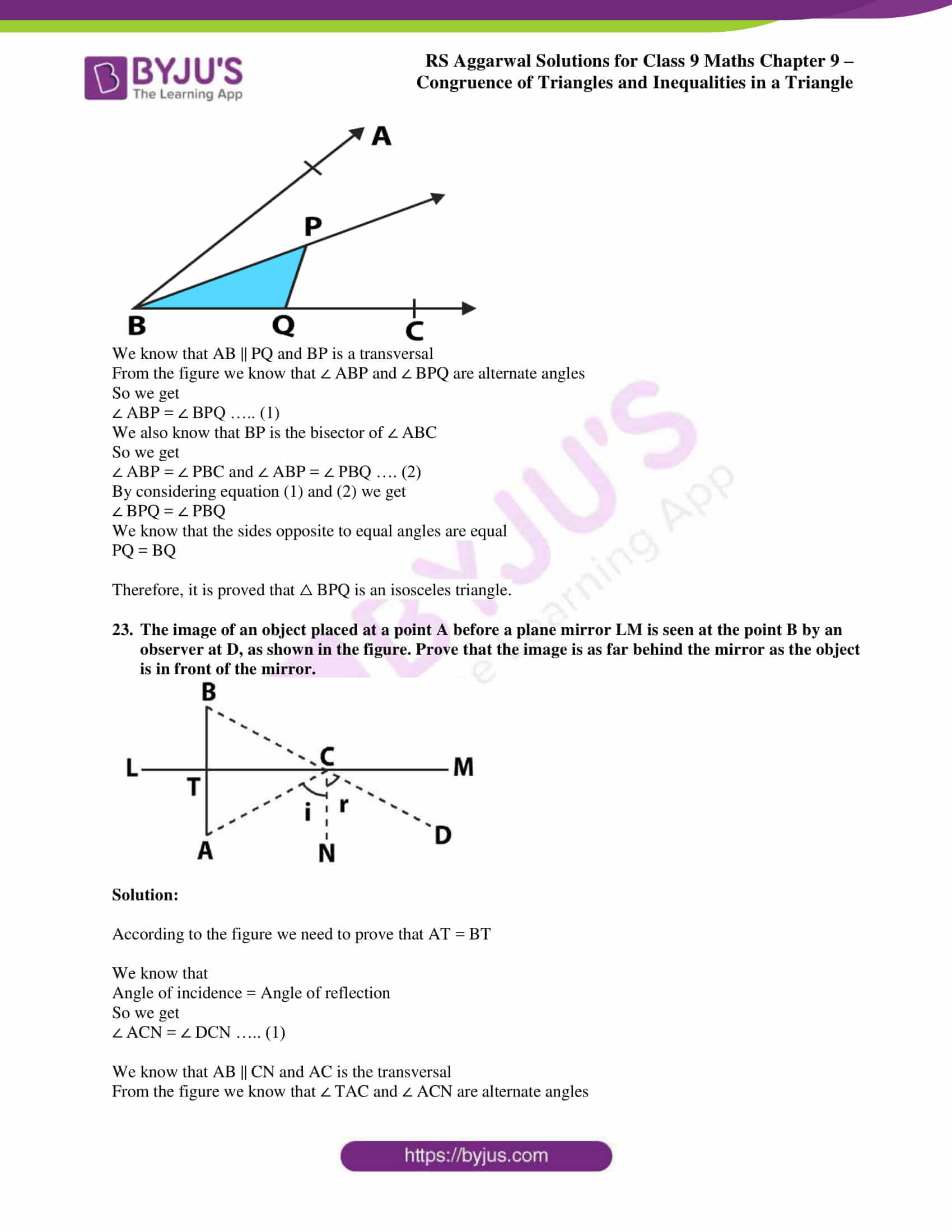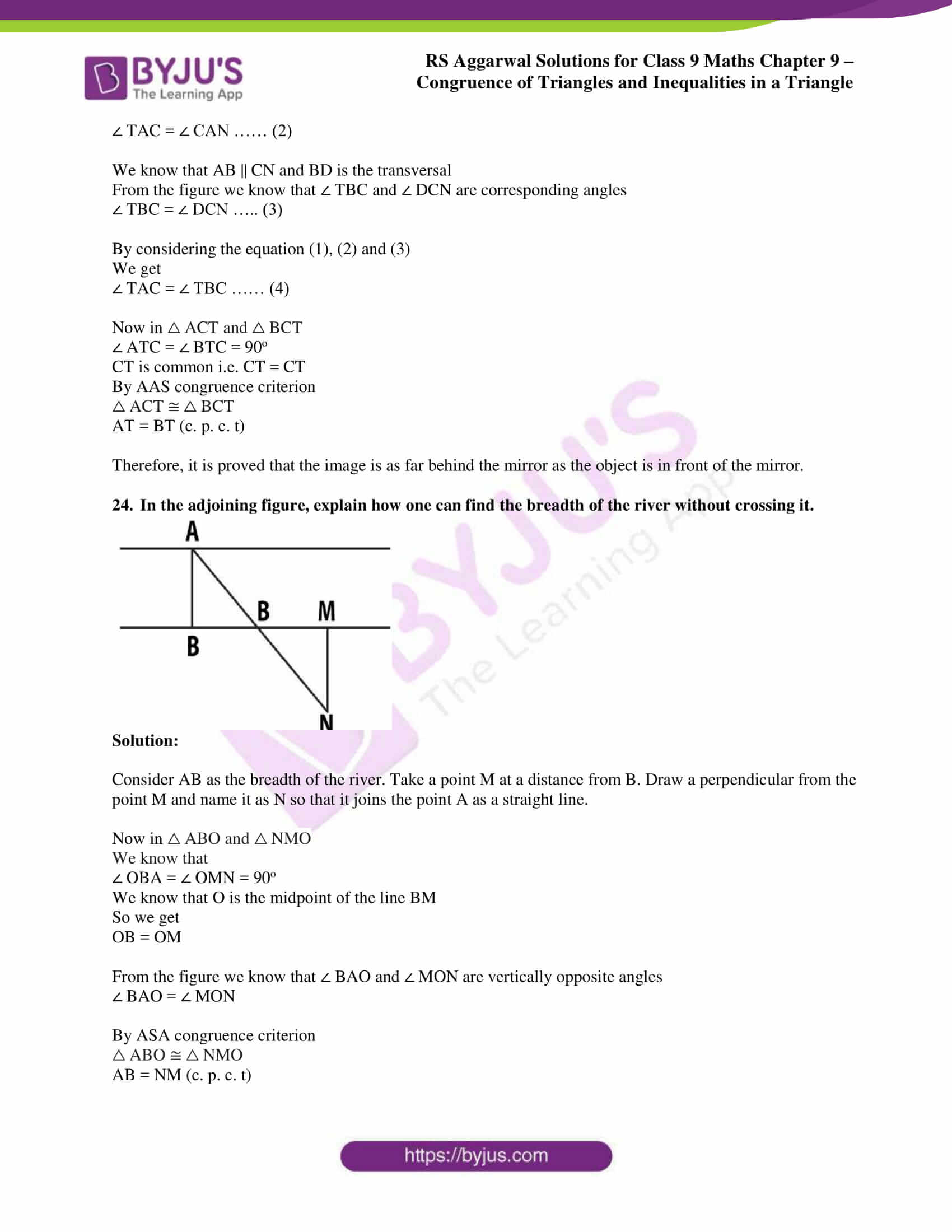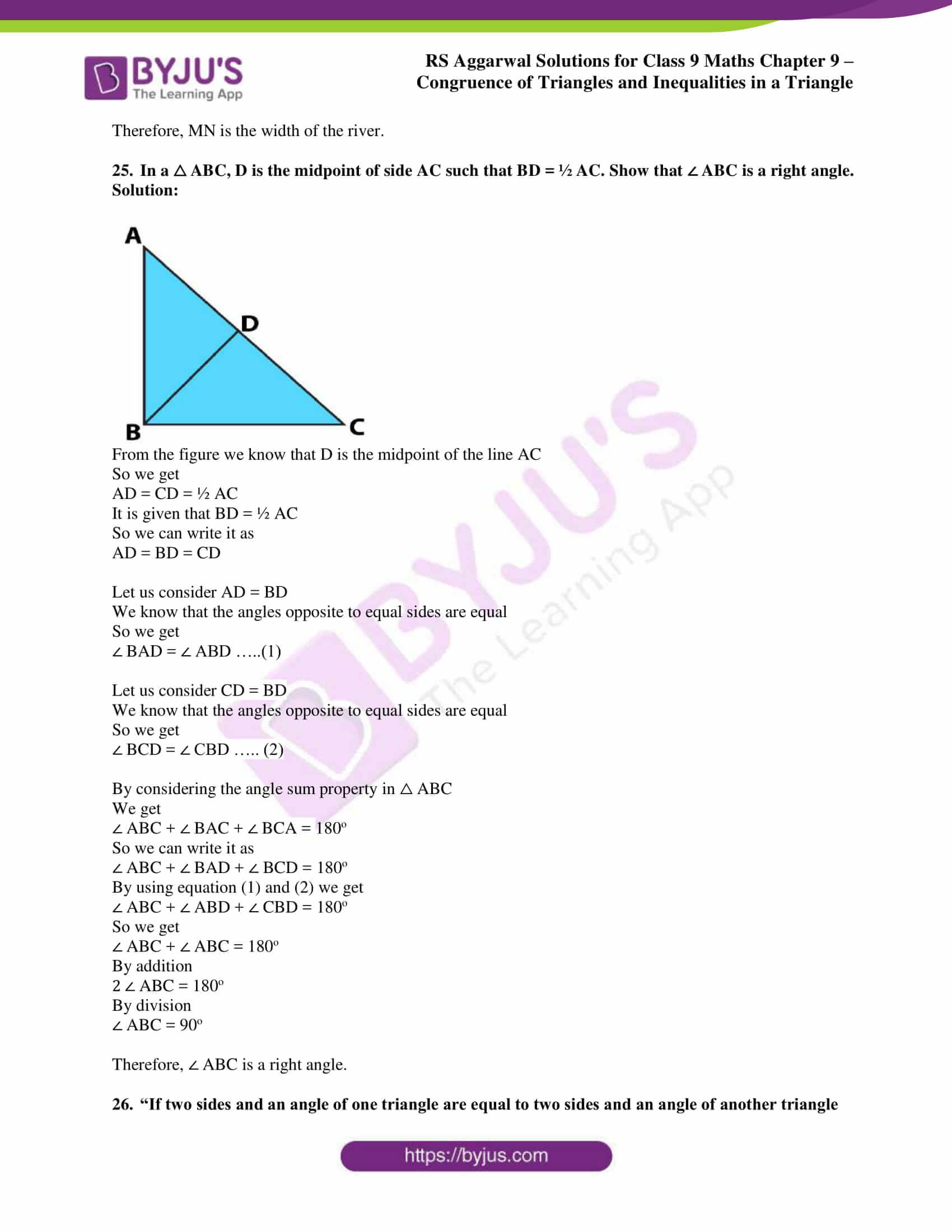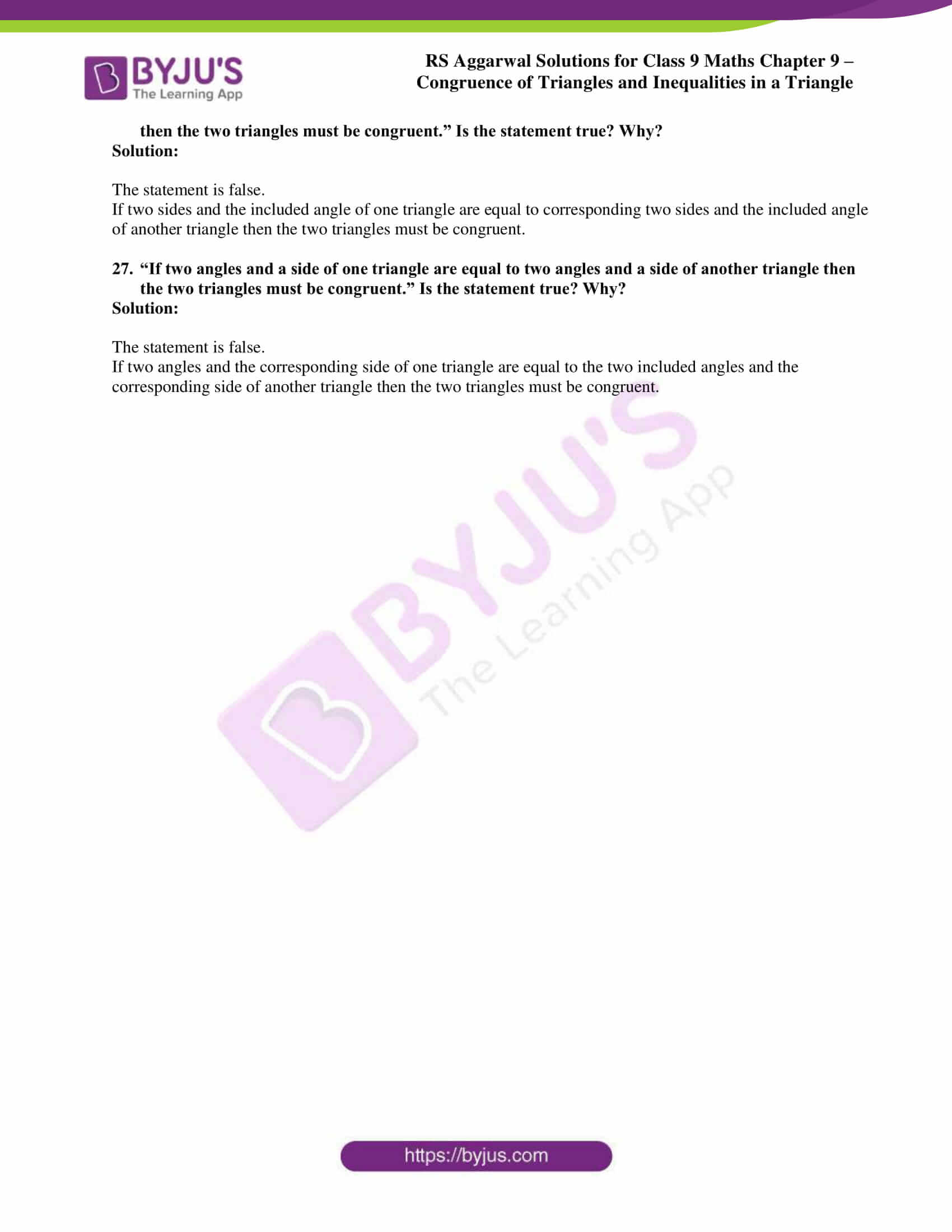## Access RS Aggarwal Solutions for Class 9 Chapter 9: Congruence of Triangles and Inequalities in a Triangle Exercise 9A

1. In the given figure, AB || CD and O is the midpoint of AD. Show that

(i) △ AOB ≅ △ DOC

(ii) O is the midpoint of BC.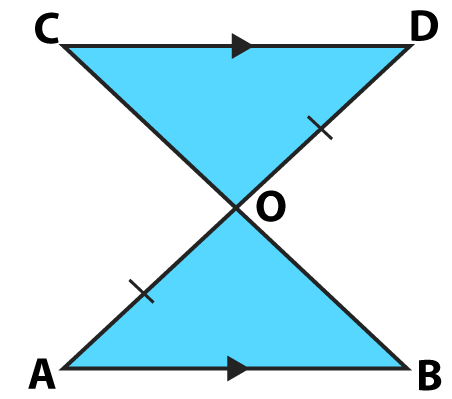Solution:

(i) From the figure △ AOB and △ DOC

We know that AB || CD and ∠ BAO and ∠ CDO are alternate angles

So we get

∠ BAO = ∠ CDO

From the figure we also know that O is the midpoint of the line AD

We can write it as AO = DO

According to the figure we know that ∠ AOB and ∠ DOC are vertically opposite angles.

So we get ∠ AOB = ∠ DOC

Therefore, by ASA congruence criterion we get

△ AOB ≅ △ DOC

(ii) We know that △ AOB ≅ △ DOC

So we can write it as

BO = CO (c. p. c. t)

Therefore, it is proved that O is the midpoint of BC.

2. In the given figure, AD and BC are equal perpendiculars to a line segment AB. Show that CD bisects AB.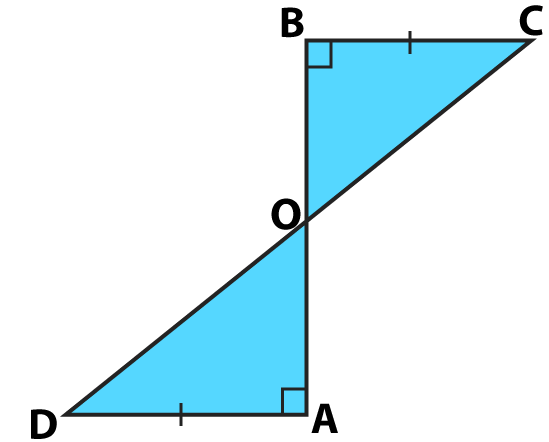Solution:

Based on the △ AOD and △ BOC

From the figure we know that ∠ AOD and ∠ BOC are vertically opposite angles.

So we get

∠ AOD = ∠ BOC

We also know that

∠ DAO = ∠ CBO = 90o

It is given that AD = BC

Therefore, by AAS congruence criterion we get

△ AOD ≅ △ BOC

So we get AO = BO (c. p. c. t)

Therefore, it is proved that CD bisects AB.

3. In the given figure, two parallel lines l and m are intersected by two parallel lines p and q. Show that △ ABC ≅ △ CDA.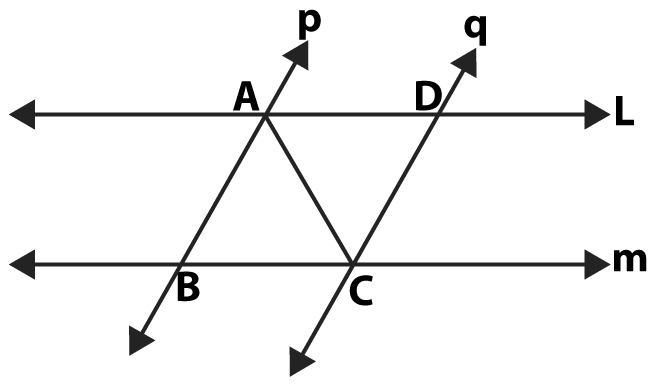Solution:

Based on the △ ABC and △ CDA

We know that p || q and ∠ BAC and ∠ DCA are alternate interior angles

So we get

∠ BAC = ∠ DCA

We know that A and C are the common points for all the lines

So it can be written as

AC = CA

We know that l || m and ∠ BCA and ∠ DAC are alternate interior angles

So we get

∠ BCA = ∠ DAC

Therefore, by ASA congruence rule it is proved that

△ ABC ≅ △ CDA

4. AD is an altitude of an isosceles △ ABC in which AB = AC. Show that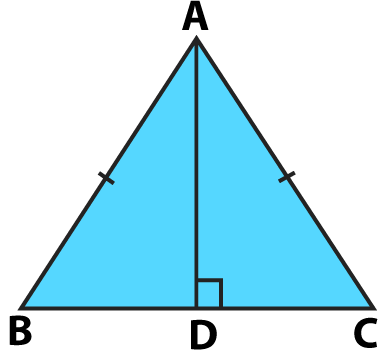Solution:

We know that AD is the altitude so the angle is 90o

So we get

It is given that AB = AC and we know that AD is common

Based on the RHS Congruence Criterion we get

So we get BD = CD (c. p. c. t)

Therefore, it is proved that AD bisects BC.

(ii) We also know that ∠ BAD = ∠ CAD (c. p. c. t)

Therefore, it is proved that AD bisects ∠ A.

5. In the given figure, BE and CF are two equal altitudes of △ ABC. Show that

(i) △ ABE ≅ △ ACF,

(ii) AB = AC.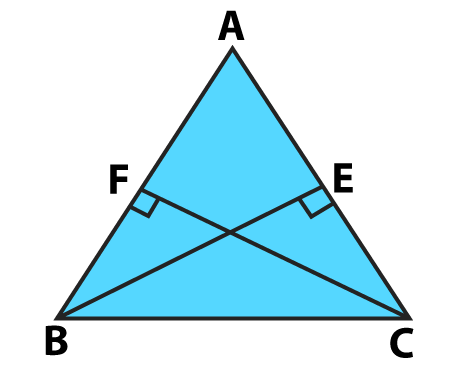Solution:

(i) Based on the △ ABE and △ ACF

We know that

∠ AEB = ∠ AFC = 90o

It is given that BE = CF

From the figure we know that ∠ A is common for both ∠ BAE and ∠ CAF

So we get

∠ BAE = ∠ CAF = ∠ A

Therefore, by ASA congruence criterion we get

△ ABE ≅ △ ACF

(ii) As we know that △ ABE ≅ △ ACF

It is proved that AB = AC.

6. △ ABC and △ DBC are two isosceles triangles on the same base BC and vertices A and D are on the same side of BC. If AD is extended to interest BC at E, show that

(i) △ ABD ≅ △ ACD

(ii) △ ABE ≅ △ ACE

(iii)AE bisects ∠ A as well as ∠ D

(iv) AE is the perpendicular bisector of BC.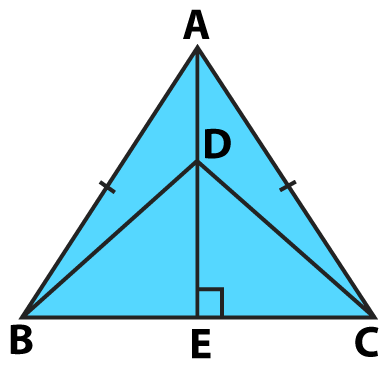Solution:

(i) Based on the △ ABD and △ ACD

From △ ABC we know that AB and AC are equal sides of isosceles triangle

So we get

AB = AC

From △ DBC we know that DB and DC are equal sides of isosceles triangle

So we get

DB = DC

Therefore, by SSS congruence criterion we get

△ ABD ≅ △ ACD

(ii) We know that △ ABD ≅ △ ACD

It can be written as

∠ BAE = ∠ CAE ……… (1)

Considering △ ABE and △ ACE

We know that AB and AC are the equal sides of isosceles △ ABC

AB = AC

So by using equation (1) we get

∠ BAE = ∠ CAE

We know that AE is common i.e. AE = AE

Therefore, by SAS congruence criterion we get

△ ABE ≅ △ ACE

(iii) We know that △ ABD ≅ △ ACD

It can be written as

∠ BAE = ∠ CAE

Therefore, it is proved that AE bisects ∠ A.

Considering △ BDE and △ CDE

We know that BD and CD are equal sides of isosceles △ ABC

Since △ ABE ≅ △ ACE

BE = CE (c. p. c. t)

We know that DE is common i.e. DE = DE

Therefore, by SSS congruence criterion we get

△ BDE ≅ △ CDE

We know that ∠ BDE = ∠ CDE (c. p. c. t)

So DE bisects ∠ D which means that AE bisects ∠ D

Hence it is proved that AE bisects ∠ A as well as ∠ D.

(iv) We know that △ BDE ≅ △ CDE

So we get

BE = CE and ∠ BED = ∠ CED (c. p. c. t)

From the figure we know that ∠ BED and ∠ CED form a linear pair of angles

So we get

∠ BED = ∠ CED = 90o

We know that DE is the perpendicular bisector of BC

Therefore, it is proved that AE is the perpendicular bisector of BC.

7. In the given figure, if x = y and AB = CB then prove that AE = CD.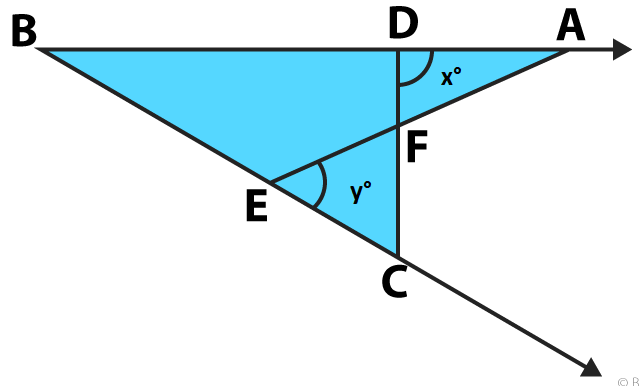Solution:

It is given that x = y and AB = CB

By considering the △ ABE

We know that

Exterior ∠ AEB = ∠ EBA + ∠ BAE

By substituting ∠ AEB as y we get

y = ∠ EBA + ∠ BAE

By considering the △ BCD

We know that

x = ∠ CBA + ∠ BCD

It is given that x = y

So we can write it as

∠ CBA + ∠ BCD = ∠ EBA + ∠ BAE

On further calculation we can write it as

∠ BCD = ∠ BAE

Based on both △ BCD and △ BAE

We know that B is the common point

It is given that AB = BC

It is proved that ∠ BCD = ∠ BAE

Therefore, by ASA congruence criterion we get

△ BCD ≅ △ BAE

We know that the corresponding sides of congruent triangles are equal

Therefore, it is proved that AE = CD.

8. In the given figure, line l is the bisector of an angle ∠ A and B is any point on l. If BP and BQ are perpendiculars from B to the arms of ∠ A, show that

(i) △ APB ≅ △ AQB

(ii) BP = BQ, i.e., B is equidistant from the arms of ∠ A.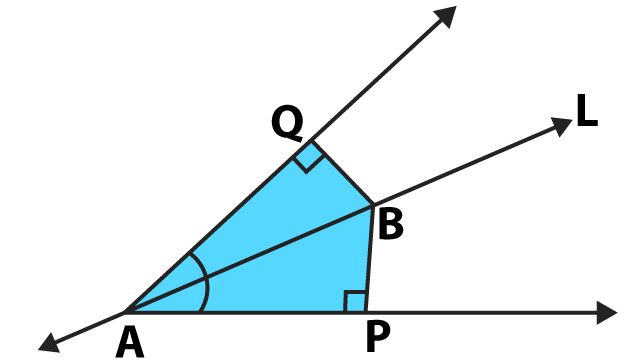Solution:

(i) Considering △ APB and △ AQB

We know that

∠ APB = ∠ AQC = 90o

From the figure we know that l is the bisector of ∠ A

So we get

∠ BAP = ∠ BAQ

We know that AB is common i.e. AB = AB

Therefore, by AAS congruence criterion we get

△ APB ≅ △ AQB

(ii) We know that △ APB ≅ △ AQB

So it is proved that BP = BQ (c. p. c. t)

9. ABCD is a quadrilateral such that diagonal AC bisects the angles ∠ A and ∠ C. Prove that AB = AD and CB = CD.

Solution: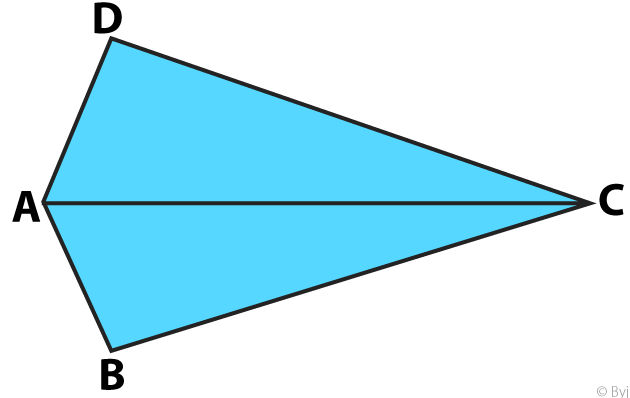By considering △ ABC and △ ADC

We know that AC bisects at ∠ A

So we get

∠ BAC = ∠ DAC

We know that AC is common i.e. AC = AC

From the figure we know that AC bisects at ∠ C

∠ BCA = ∠ DCA

By ASA congruence criterion we get

Therefore, it is proved that AB = AD and CB = CD (c. p. c. t)

10. △ ABC is a right triangle right angled at A such that AB = AC and bisector of ∠ C intersects the side AB at D. Prove that AC + AD = BC.

Solution: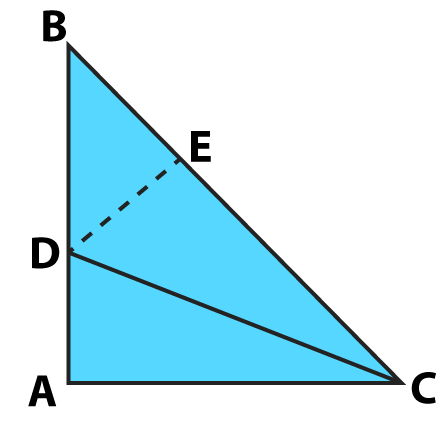Construct a triangle ABC where DE is perpendicular to BC

Consider the △ DAC and △ DEC

We know that

∠ BAC = ∠ DAC = 90o

From the figure we know that CD bisects ∠ C

So we get

∠ DCA = ∠ DCE

We know that CD is common i.e. CD = CD

By AAS congruence criterion

△ DAC ≅ △ DEC

So we know that DA = DE …… (1)

AC = EC (c. p. c. t) ….. (2)

It is given that AB = AC

We know that the angles opposite to equal sides are equal

∠ B = ∠ C

We know that the sum of angles of △ ABC is 180o.

∠ A + ∠ B + ∠ C = 180o

By substituting the values

90o + ∠ B + ∠ B = 180o

On further calculation

2 ∠ B = 180o – 90o

2 ∠ B = 90o

By division

∠ B = 45o

Considering the △ BED

We know that ∠ BED = 90o

So we can write it as

∠ BDE + ∠ B = 90o

By substituting the values

∠ BDE + 45o = 90o

On further calculation

∠ BDE = 90o – 45o

By subtraction

∠ BDE = 45o

It can be written as

∠ BDE = ∠ DBE = 45o

We know that DE and BE are the equal sides of isosceles triangle

DE = BE …….. (3)

By comparing the equations (1) and (3)

We get

DA = DE = BE …… (4)

We know that BC = BE + EC

By considering the equations (ii) and (iv)

We get

BC = DA + AC

We can also write it as

Therefore, it is proved that AC + AD = BC.

11. In the given figure, OA = OB and OP = OQ. Prove that

(i) PX = QX,

(ii) AX = BX.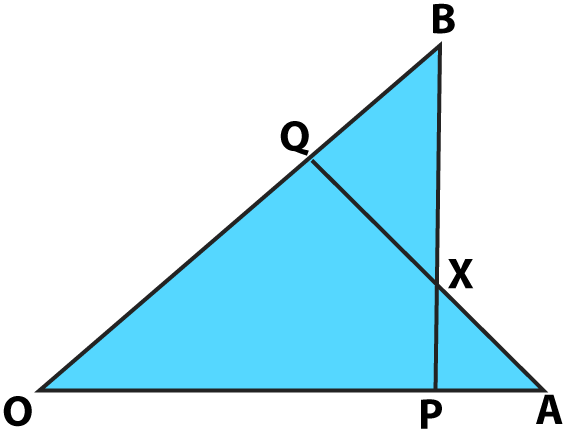Solution:

It is given that OA = OB and OP = OQ

By considering the △ OAQ and △ OPB

Therefore, by SAS congruence criterion

△ OAQ = △ OPB

We know that the corresponding parts of congruent triangles are equal

So we get

∠ OBP = ∠ OAQ …….. (1)

Consider △ BXQ and △ PXA

We can write it as

BQ = OB – OQ and PA = OA – OP

We know that OP = OQ and is given that OA = OB

So we get BQ = PA ……. (2)

In △ BXQ and △ PXA

We know that ∠ BXQ and ∠ PXA are vertically opposite angles

∠ BXQ = ∠ PXA

From (1) and (2) and AAS congruence criterion we get

△ BXQ ≅ △ PXA

So we get PX = QX and AX = BX (c. p. c. t)

12. In the given figure, ABC is an equilateral triangle; PQ || AC and AC is produced to R such that CR = BP. Prove that QR bisects PC.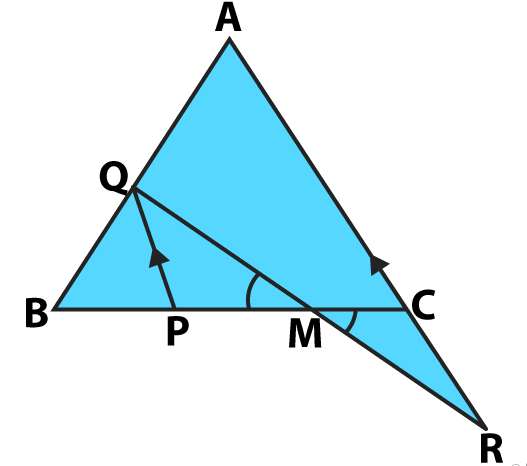Solution:

It is given that ABC is an equilateral triangle; PQ || AC and AC is produced to R such that CR = BP

Consider QR intersecting the line PC at point M

We know that △ ABC is an equilateral triangle

So we get ∠ A = ∠ ACB = 60o

From the figure we know that PQ || AC and ∠ BPQ and ∠ ACB are corresponding angles

So we get

∠ BPQ = ∠ ACB = 60o

Based on the △ BPQ we know that

∠ B = ∠ ACB = 60o

It can be written as

∠ BQP = 60o

According to the figure we know that △ BPQ is an equilateral triangle

So we get

PQ = BP = BQ

It is given that CR = BP so we get

PQ = CR ……. (1)

In the △ PMQ and △ CMR we know that PQ || AC and QR is the transversal

We know that ∠ PQM and ∠ CRM are alternate angles and ∠ PMQ and ∠ CMR are vertically opposite angles

∠ PQM = ∠ CRM

∠ PMQ = ∠ CMR

By considering equation (1) and AAS congruence criterion

△ PMQ ≅ △ CMR

We know that the corresponding parts of congruent triangles are equal

PM = MC

Therefore, it is proved that QR bisects PC.

13. In the given figure, ABCD is a quadrilateral in which AB || DC and P is the midpoint of BC. On producing, AP and DC meet at Q. Prove that

(i) AB = CQ,

(ii) DQ = DC + AB.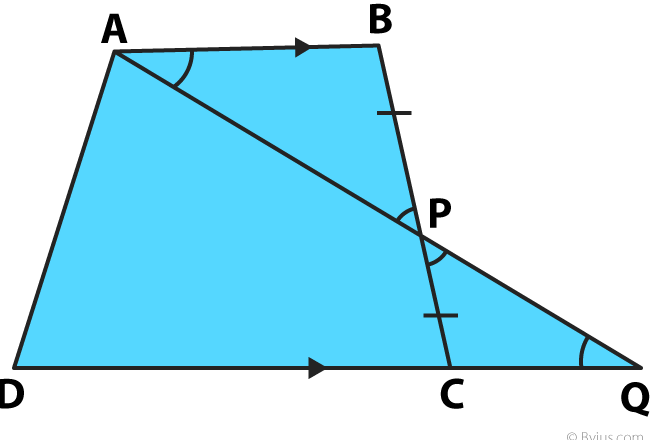Solution:

It is given that

ABCD is a quadrilateral in which AB || DC and P is the midpoint of BC

Considering △ ABP and △ PCQ

We know that ∠ PAB and ∠ PQC are alternate angles and ∠ APB and ∠ CPQ are vertically opposite angles

∠ PAB = ∠ PQC

∠ APB = ∠ CPQ

According to AAS congruence criterion

△ ABP ≅ △ PCQ

AB = CQ (c. p. c. t) …… (i)

We know that

DQ = DC + CQ

By substituting CQ as AB we get

DQ = DC + AB …… (ii)

Therefore, it is proved that AB = CQ and DQ = DC + AB.

14. In the given figure, ABCD is a square and P is a point inside it such that PB = PD. Prove that CPA is a straight line.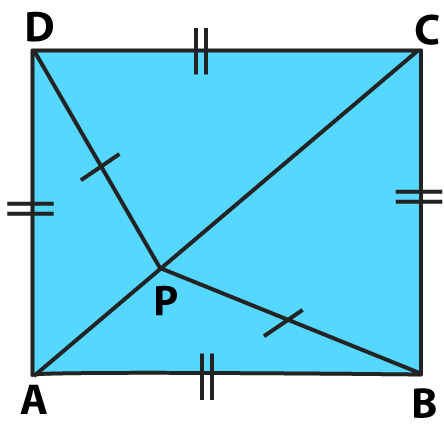Solution:

It is given that ABCD is a square and P is a point inside it such that PB = PD

Considering △ APD and △ APB

We know that all the sides are equal in a square

So we get DA = AB

AP is common i.e. AP = AP

According to SSS congruence criterion

△ APD ≅ △ APB

We get ∠ APD = ∠ APB (c. p. c. t)….. (1)

Considering △ CPD and △ CPB

We know that all the sides are equal in a square

So we get CD = CB

CP is common i.e. CP = CP

According to SSS congruence criterion

△ CPD ≅ △ CPB

We get ∠ CPD = ∠ CPB (c. p. c. t)….. (2)

By adding both the equation (1) and (2)

∠ APD + ∠ CPD = ∠ APB + ∠ CPB ……. (3)

From the figure we know that the angles surrounding the point P is 360o

So we get

∠ APD + ∠ CPD + ∠ APB + ∠ CPB = 360o

By grouping we get

∠ APB + ∠ CPB = 360o – (∠ APD + ∠ CPD) …… (4)

Now by substitution of (4) in (3)

∠ APD + ∠ CPD = 360o – (∠ APD + ∠ CPD)

On further calculation

2 (∠ APD + ∠ CPD) = 360o

By division we get

∠ APD + ∠ CPD = 180o

Therefore, it is proved that CPA is a straight line.

15. In the given figure, O is a point in the interior of square ABCD such that △ OAB is an equilateral triangle. Show that △ OCD is an isosceles triangle.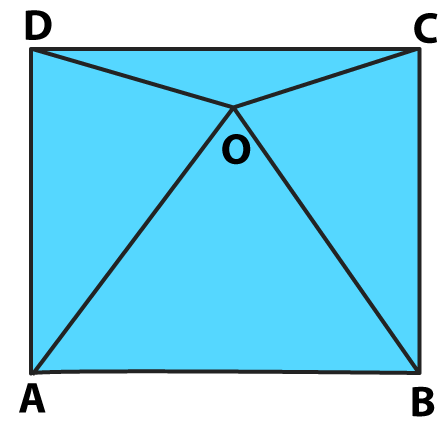Solution:

We know that △ OAB is an equilateral triangle

So it can be written as

∠ OAB = ∠ OBA = AOB = 60o

From the figure we know that ABCD is a square

So we get

∠ A = ∠ B = ∠ C = ∠ D = 90o

In order to find the value of ∠ DAO

We can write it as

∠ A = ∠ DAO + ∠ OAB

By substituting the values we get

90o = ∠ DAO + 60o

On further calculation

∠ DAO = 90o – 60o

By subtraction

∠ DAO = 30o

We also know that ∠ CBO = 30o

Considering the △ OAD and △ OBC

We know that the sides of a square are equal

We know that the sides of an equilateral triangle are equal

OA = OB

By SAS congruence criterion

So we get OD = OC (c. p. c. t)

Therefore, it is proved that △ OCD is an isosceles triangle.

16. In the adjoining figure, X and Y are respectively two points on equal sides AB and AC of △ ABC such that AX = AY. Prove that CX = BY.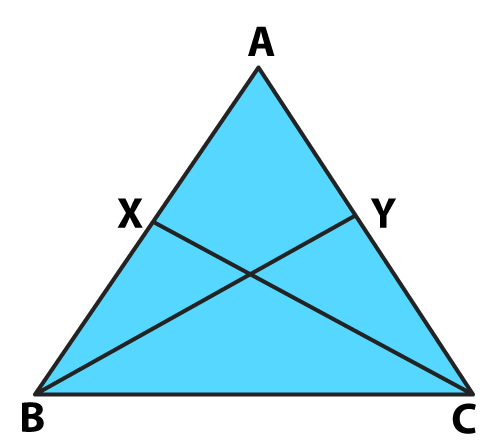Solution:

It is given that AX = AY

Considering △ AXC and △ AYB

∠ A is common i.e. ∠ A = ∠ A

We know that the sides of equilateral triangle are equal so we get

AC = AB

By SAS congruence criterion

△ AXC ≅ △ AYB

XC = YB (c. p. c. t)

Therefore, it is proved that CX = BY.

17. In △ ABC, D is the midpoint of BC. If DL ⊥ AB and DM ⊥ AC such that DL = DM, prove that AB =AC.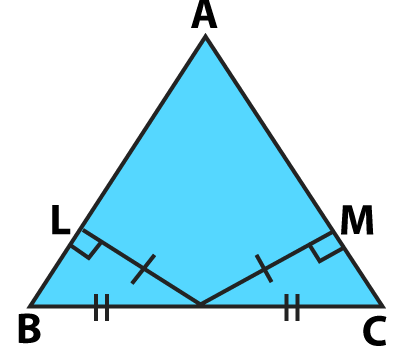Solution:

It is given that D is the midpoint of BC

DL ⊥ AB and DM ⊥ AC such that DL = DM

Considering △ BLD and △ CMD as right angled triangle

So we can write it as

∠ BLD = ∠ CMD = 90o

We know that BD = CD and DL = DM

By RHS congruence criterion

△ BLD = △ CMD

∠ ABD = ∠ ACD (c. p. c. t)

Now, in △ ABC

∠ ABD = ∠ ACD

We know that the sides opposite to equal angles are equal so we get

AB = AC

Therefore, it is proved that AB = AC.

18. In △ ABC, AB = AC and the bisectors ∠ B and ∠ C meet at a point O. Prove that BO = CO and the ray AO is the bisector of ∠ A.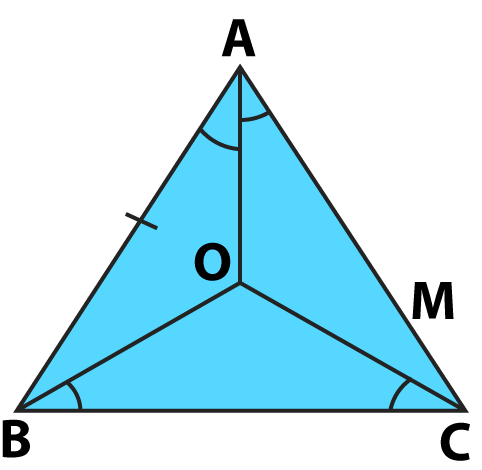Solution:

It is given that AB = AC and the bisectors ∠ B and ∠ C meet at a point O

Consider △ BOC

So we get

∠ BOC = ½ ∠ B and ∠ OCB = ½ ∠ C

It is given that AB = AC so we get ∠ B = ∠ C

So we get

∠ OBC = ∠ OCB

We know that if the base angles are equal even the sides are equal

So we get OB = OC ……. (1)

∠ B and ∠ C has the bisectors OB and OC so we get

∠ ABO = ½ ∠ B and ∠ ACO = ½ ∠ C

So we get

∠ ABO = ∠ ACO …….. (2)

Considering △ ABO and △ ACO and equation (1) and (2)

It is given that AB = AC

By SAS congruence criterion

△ ABO ≅ △ ACO

∠ BAO = ∠ CAO (c. p. c. t)

Therefore, it is proved that BO = CO and the ray AO is the bisector of ∠ A.

19. The line segments joining the midpoints M and N are parallel sides AB and DC respectively of a trapezium ABCD is perpendicular to both the sides AB and DC. Prove that AD = BC.

Solution:

Construct AN and BN at the point N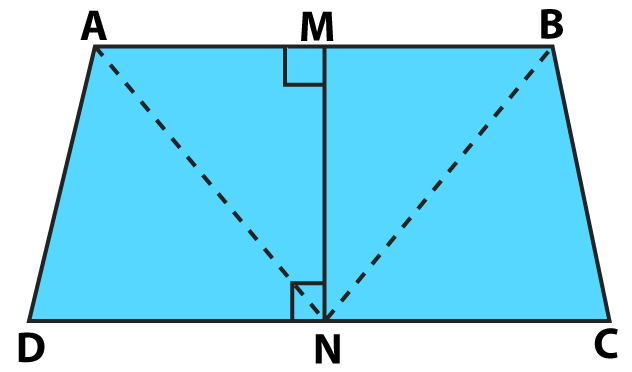Consider △ ANM and △ BNM

We know that N is the midpoint of the line AB

So we get

AM = BM

From the figure we know that

∠ AMN = ∠ BMN = 90o

MN is common i.e. MN = MN

By SAS congruence criterion

△ ANM ≅ △ BNM

AN = BN (c. p. c. t) …… (1)

We know that

∠ ANM = ∠ BNM (c. p. c. t)

Subtracting LHS and RHS by 90o

90o – ∠ ANM = 90o – ∠ BNM

So we get

∠ AND = ∠ BNC …… (2)

Now, consider △ AND and △ BNC

AN = BN

∠ AND = ∠ BNC

We know that N is the midpoint of the line DC

DN = CN

By SAS congruence criterion

△ AND ≅ △ BNC

AD = BC (c. p. c. t)

Therefore, it is proved that AD = BC.

20. The bisectors ∠ B and ∠ C of and isosceles triangle with AB = AC intersect each other at a point O. BO is produced to meet AC at a point M. Prove that ∠ MOC = ∠ ABC.

Solution: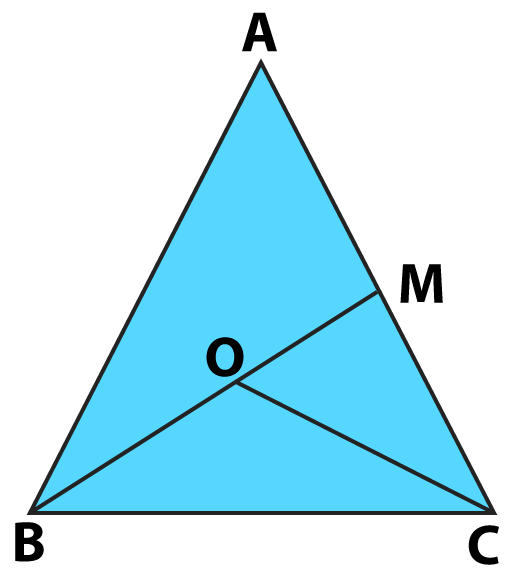Consider △ ABC

It is given that AB = AC

So we get

∠ ABC = ∠ ACB

Dividing both sides by 2 we get

½ ∠ ABC = ½ ∠ ACB

So we get

∠ OBC = ∠ OCB

By using the exterior angle property

We get

∠ MOC = ∠ OBC + ∠ OCB

We know that ∠ OBC = ∠ OCB

So we get

∠ MOC = 2 ∠ OBC

We know that OB is the bisector of ∠ ABC

∠ MOC = ∠ ABC

Therefore, it is proved that ∠ MOC = ∠ ABC.

21. The bisectors of ∠ B and ∠ C of an isosceles △ ABC with AB = AC intersect each other at a point O. Show that the exterior angle adjacent to ∠ ABC is equal to ∠ BOC.

Solution: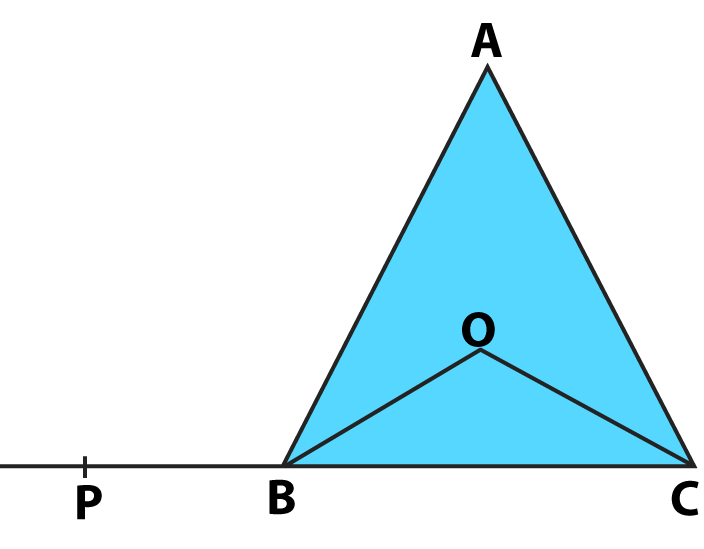Considering the △ ABC

It is given that AB = AC

So we get

∠ ABC = ∠ ACB

Dividing by 2 both sides

½ ∠ ABC = ½ ∠ ACB

So we get

∠ OBC = ∠ OCB …… (1)

By using the angle sum property in △ BOC

∠ BOC + ∠ OBC + ∠ OCB = 180o

Substituting equation (1)

∠ BOC + 2 ∠ OBC = 180o

So we get

∠ BOC + ∠ ABC = 180o

From the figure we know that ∠ ABC and ∠ ABP form a linear pair of angles so we get

∠ ABC + ∠ ABP = 180o

∠ ABC = 180o – ∠ ABP

By substituting the value in the above equation we get

∠ BOC + (180o – ∠ ABP) = 180o

On further calculation

∠ BOC + 180o – ∠ ABP = 180o

By subtraction

∠ BOC – ∠ ABP = 180o – 180o

∠ BOC – ∠ ABP = 0

∠ BOC = ∠ ABP

Therefore, it is proved that the exterior angle adjacent to ∠ ABC is equal to ∠ BOC.

22. P is a point on the bisector of ∠ ABC. If the line through P, parallel to BA meets BC at Q, prove that △ BPQ is an isosceles triangle.

Solution: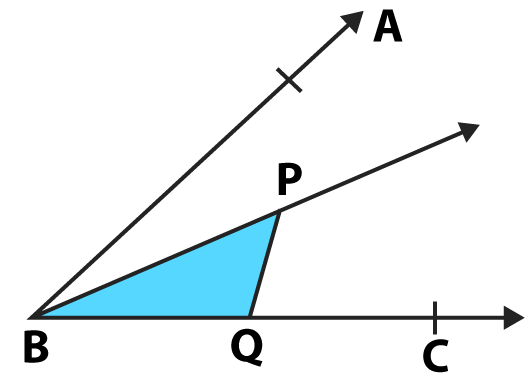We know that AB || PQ and BP is a transversal

From the figure we know that ∠ ABP and ∠ BPQ are alternate angles

So we get

∠ ABP = ∠ BPQ ….. (1)

We also know that BP is the bisector of ∠ ABC

So we get

∠ ABP = ∠ PBC and ∠ ABP = ∠ PBQ …. (2)

By considering equation (1) and (2) we get

∠ BPQ = ∠ PBQ

We know that the sides opposite to equal angles are equal

PQ = BQ

Therefore, it is proved that △ BPQ is an isosceles triangle.

23. The image of an object placed at a point A before a plane mirror LM is seen at the point B by an observer at D, as shown in the figure. Prove that the image is as far behind the mirror as the object is in front of the mirror.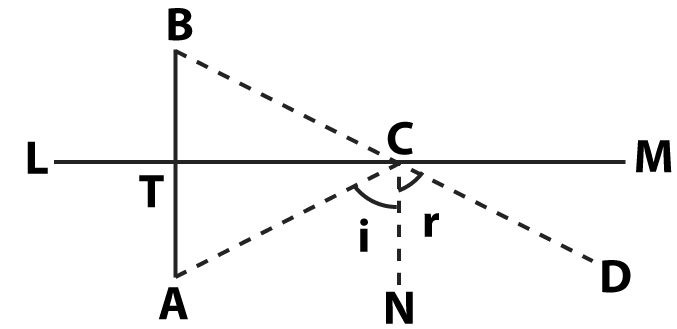Solution:

According to the figure we need to prove that AT = BT

We know that

Angle of incidence = Angle of reflection

So we get

∠ ACN = ∠ DCN ….. (1)

We know that AB || CN and AC is the transversal

From the figure we know that ∠ TAC and ∠ ACN are alternate angles

∠ TAC = ∠ CAN …… (2)

We know that AB || CN and BD is the transversal

From the figure we know that ∠ TBC and ∠ DCN are corresponding angles

∠ TBC = ∠ DCN ….. (3)

By considering the equation (1), (2) and (3)

We get

∠ TAC = ∠ TBC …… (4)

Now in △ ACT and △ BCT

∠ ATC = ∠ BTC = 90o

CT is common i.e. CT = CT

By AAS congruence criterion

△ ACT ≅ △ BCT

AT = BT (c. p. c. t)

Therefore, it is proved that the image is as far behind the mirror as the object is in front of the mirror.

24. In the adjoining figure, explain how one can find the breadth of the river without crossing it.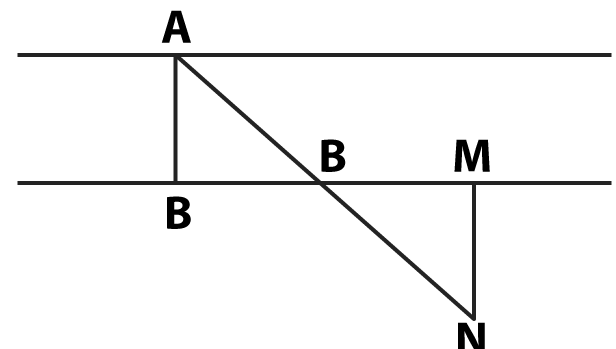Solution:

Consider AB as the breadth of the river. Take a point M at a distance from B. Draw a perpendicular from the point M and name it as N so that it joins the point A as a straight line.

Now in △ ABO and △ NMO

We know that

∠ OBA = ∠ OMN = 90o

We know that O is the midpoint of the line BM

So we get

OB = OM

From the figure we know that ∠ BAO and ∠ MON are vertically opposite angles

∠ BAO = ∠ MON

By ASA congruence criterion

△ ABO ≅ △ NMO

AB = NM (c. p. c. t)

Therefore, MN is the width of the river.

25. In a △ ABC, D is the midpoint of side AC such that BD = ½ AC. Show that ∠ ABC is a right angle.

Solution: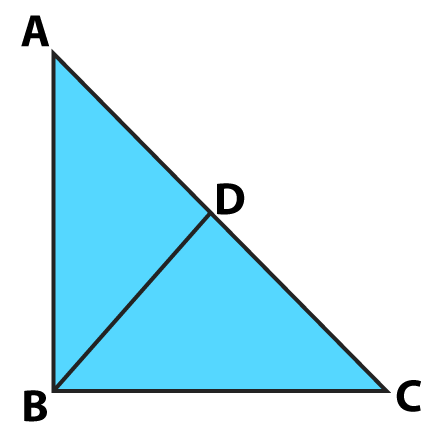From the figure we know that D is the midpoint of the line AC

So we get

AD = CD = ½ AC

It is given that BD = ½ AC

So we can write it as

Let us consider AD = BD

We know that the angles opposite to equal sides are equal

So we get

∠ BAD = ∠ ABD …..(1)

Let us consider CD = BD

We know that the angles opposite to equal sides are equal

So we get

∠ BCD = ∠ CBD ….. (2)

By considering the angle sum property in △ ABC

We get

∠ ABC + ∠ BAC + ∠ BCA = 180o

So we can write it as

∠ ABC + ∠ BAD + ∠ BCD = 180o

By using equation (1) and (2) we get

∠ ABC + ∠ ABD + ∠ CBD = 180o

So we get

∠ ABC + ∠ ABC = 180o

2 ∠ ABC = 180o

By division

∠ ABC = 90o

Therefore, ∠ ABC is a right angle.

26. “If two sides and an angle of one triangle are equal to two sides and an angle of another triangle then the two triangles must be congruent.” Is the statement true? Why?

Solution:

The statement is false.

If two sides and the included angle of one triangle are equal to corresponding two sides and the included angle of another triangle then the two triangles must be congruent.

27. “If two angles and a side of one triangle are equal to two angles and a side of another triangle then the two triangles must be congruent.” Is the statement true? Why?

Solution:

The statement is false.

If two angles and the corresponding side of one triangle are equal to the two included angles and the corresponding side of another triangle then the two triangles must be congruent.

### Access other exercise solutions of Class 9 Maths Chapter 9: Congruence of Triangles and Inequalities in a Triangle

Exercise 9B Solutions 16 Questions

### RS Aggarwal Solutions Class 9 Maths Chapter 9 – Congruence of Triangles and Inequalities in a Triangle Exercise 9A

RS Aggarwal Solutions Class 9 Maths Chapter 9 Congruence of Triangles and Inequalities in a Triangle Exercise 9A has problems solved using congruence criteria like SAS, ASA, AAS, SSS and RHS.

### Key features of RS Aggarwal Solutions for Class 9 Maths Chapter 9: Congruence of Triangles and Inequalities in a Triangle Exercise 9A

• RS Aggarwal Solutions help students to revise the entire syllabus faster with a better conceptual knowledge.
• The stepwise explanations in each problem boosts confidence among students to perform well in the exam.
• The solutions are prepared according to the CBSE guidelines to make the students familiar with the concepts weightage.
• RS Aggarwal solutions contain problems which are frequently asked in the board exam to improve confidence among students.